## Algorithms

 Question 1
The following paradigm can be used to find the solution of the problem in minimum time: Given a set of non-negative integer, and a value K, determine if there is a subset of the given set with the sum equal to K:
 A Divide and Conquer B Dynamic Programming C Greedy Algorithm D Branch and Bound
Algorithms       Dynamic-Programming       ISRO-2018
Question 1 Explanation:
The above problem clearly specifies that sum of subset problem. The sum of the subset problem using recursion will give exponential time. Using dynamic programming we can get pseudo-polynomial time.
Sum of subset problem:
Given a set of non-negative integers, and a value sum, determine if there is a subset of the given set with the sum equal to the given sum.

Example:
Array set[ ]= {1,2,3,4,5} and sum=6
Here there are possible subsets (2,4),(1,2,3) and (5,1).
 Question 2
Given two sorted list of size m and n respectively. The number of comparisons needed the worst case by the merge sort algorithm will be
 A m*n B maximum of m and n C minimum of m and n D m+n–1
Algorithms       Sorting       ISRO-2018
Question 2 Explanation:
Here the maximum number of comparisons is m+n-1.
For example: Take 2 sub-arrays of size 3 such as 1,3,5 and another subarray 2,4,6. Then in this case number of comparisons is 5 which is m+n-1 can be taken as O(m+n).
 Question 3
Of the following sorting algorithms, which has a running time that is least dependent on the initial ordering of the input?
 A Merge Sort B Insertion Sort C Selection Sort D Quick Sort
Algorithms       Sorting       ISRO-2018
Question 3 Explanation:Question 4
The running time of an algorithm is given byThen what should be the relation between T(1), T(2) and T(3), so that the order of the algorithm is constant?
 A T(1) = T(2) = T(3) B T(1) + T(3) = 2 * T(2) C T(1) – T(3) = T(2) D T(1) + T(2) = T(3)
Algorithms       Time-Complexity       ISRO-2018
Question 4 Explanation:
T(n)= T(n-1) + T(n-2) – T(n-3)"
Solution:
Better way of solving is substitute.
T(1) = 1
T(2) = 2
T(3) = 3
T(4) = T(3) + T(2) - T(1) = 4
T(5) = T(4) + T(3) - T(2) = 5
:
:
T(n) = T(n-1) + T(n-2) - T(n-3) = n-1 + n-2 - n + 3 = n
So order is O(n)
 Question 5
The time complexity of computing the transitive closure of binary relation on a set of n elements is known to be
 A O(n) B O(n log n) C O(n3/2) D O(n3)
Algorithms       Time-Complexity       ISRO-2018
Question 5 Explanation:
→ In mathematics the transitive closure of a binary relation R on a set X is defined as the smallest relation on X that contains R and is transitive. More formally, the transitive closure of a binary relation R on a set X is the transitive relation R+ on set X such that R+ contains R and R+ is minimal.
→ For example, if X is a set of airports and x R y means "there is a direct flight from airport x to airport y" (for x and y in X), then the transitive closure of R on X is the relation R+ such that x R+ y means "it is possible to fly from x to y in one or more flights". Informally, the transitive closure gives you the set of all places you can get to from any starting place.
→ To find the transitive closure of given relation, we can represent the given relation by the graph such that if x R y then there should be directed edge between x and y in a graph.
→ The time complexity of floyd warshall algorithm is O(V3) where V is the number of vertices in the given graph. Take V as the number of elements is set i.e., N.
→ Therefore time complexity for the given question is O(n3).
 Question 6
The level of aggregation of information required for operational control is
 A Detailed B Aggregate C Qualitative D None of the above
Algorithms       Aggregation       ISRO-2007
Question 6 Explanation:Question 7
Selection sort algorithm design technique is an example of
 A Greedy method B Divide-and-conquer C Dynamic Programming D Backtracking
Algorithms       Sorting       ISRO-2007
Question 7 Explanation:
The selection sort algorithm sorts an array by repeatedly finding the minimum element (considering ascending order) from unsorted part and putting it at the beginning. The algorithm maintains two subarrays in a given array.
1) The subarray which is already sorted.
2) Remaining subarray which is unsorted.
In every iteration of selection sort, the minimum element (considering ascending order) from the unsorted subarray is picked and moved to the sorted subarray. Clearly, it is a greedy approach to sort the array.
 Question 8
The average case and worst case complexities for Merge sort algorithm are
 A O(n2), O(n2) B O(n2), O(nlog2n) C O(n log2n), O(n2) D O(n log2n), O(n log2n)
Algorithms       Sorting       ISRO-2007
Question 8 Explanation:
The best case, average case and worst case complexities for Merge sort algorithm are O( nlog2n ).
 Question 9
The time taken by binary search algorithm to search a key in a sorted array of n elements is
 A O ( log2n ) B O ( n ) C O ( n log2n ) D O ( n2 )
Algorithms       Searching       ISRO-2007
Question 9 Explanation:
Search a sorted array by repeatedly dividing the search interval in half. Begin with an interval covering the whole array. If the value of the search key is less than the item in the middle of the interval, narrow the interval to the lower half. Otherwise narrow it to the upper half. Repeatedly check until the value is found or the interval is empty. It takes a maximum of log(n) searches to search an element from the sorted array.
 Question 10
The Fibonacci sequence is the sequence of integers
 A 1, 3, 5, 7, 9, 11, 13 B 0, 1, 1, 2, 3, 5, 8, 13, 21, 34, 55 C 0, 1, 3, 4, 7, 11, 18, 29, 47 D 0, 1, 3, 7, 15
Algorithms       Fibbonacci-Series       ISRO-2007
Question 10 Explanation:
In mathematical terms, the sequence Fn of Fibonacci numbers is defined by the recurrence relation Fn = Fn-1 + Fn-2
with seed values
F0 = 0 and F1 = 1.
The Fibonacci numbers are the numbers in the following integer sequence.
0, 1, 1, 2, 3, 5, 8, 13, 21, 34, 55, 89, 144..
 Question 11
The problems 3-SAT and 2-SAT are
 A Both NP-complete B Both in P C NP-complete and in P, respectively D Undecidable and NP-complete, respectively
Algorithms       NP complete       ISRO-2017 May
Question 11 Explanation:
→ 2-SAT is the first non polynomial problem is solved in polynomial time.
→ 3-SAT problem is NP- complete problem
 Question 12
The recurrence relation that arises in relation with the complexity of binary search is:
 A T(n)=2T(n/2)+k , where k is constant B T(n)=T(n/2) +k, where k is constant C T(n)=T(n/2)+logn D T(n)=T(n/2)+n
Algorithms       Searching       ISRO-2017 May
Question 12 Explanation:
Binary search in a sorted array
The time to search in an array of ‘n’ elements is equal to the time to search in an array of n/2 elements plus k comparison.
T(n)=T(n/2)+k // k is constant
 Question 13
Which one of the following in-place sorting algorithms needs the minimum number of swaps?
 A Insertion Sort B Quick Sort C Heap Sort D Selection Sort
Algorithms       Sorting       ISRO-2017 May
Question 13 Explanation:
Selection sort requires minimum number of swaps i.e O(n)Question 14
The number of swappings needed to sort the numbers 8, 22, 7, 9, 31, 5, 13 in ascending order, using bubble sort is
 A 11 B 12 C 13 D 10
Algorithms       Sorting       ISRO-2017 May
Question 14 Explanation:
Step-1: 8,7,22,9,31,5,13
Step-2: 8,7,9,22,31,5,13
Step-3: 8,7,9,22,5,31,13
Step-4: 8,7,9,22,5,13,31
Step-5: 7,8,9,22,5,13,31
Step-6: 7,8,9,5,22,13,31
Step-7: 7,8,9,5,13,22,31
Step-8: 7,8,5,9,13,22,31
Step-9: 7,5,8,9,13,22,31
Step-10: 5,7,8,9,12,22,31
Note:Total 10 swaps are required to sort the array.
 Question 15
Which of the following algorithm solves the all-pairs shortest path problem?
 A Prim’s algorithm B Dijkstra's algorithm C Bellman-Ford’s algorithm D Floyd-Warshall’s algorithm
Algorithms       Dynamic-Programming       ISRO-2017 May
Question 15 Explanation:
Prim's → Minimum Spanning tree
Dijkstra's algorithm → Single source shortest path for only positive values
Bellman Ford’s algorithm → Single source shortest path for either positive values or negative values but not negative weight cycle.
Floyd-Warshall’s algorithm→ All pair shortest path problem
 Question 16
The time complexity of computing the transitive closure of binary relation on a set of n elements is known to be
 A O(nlogn) B O(n3/2) C O(n3) D O(n)
Algorithms       Time-Complexity       ISRO-2017 May
Question 16 Explanation:
Transitive closure generally uses Floyd-Warshall Algorithm which gives a time complexity of O(n3)
 Question 17
Which one of the following algorithm design techniques is used in finding all pairs of shortest distances in a graph?
 A Dynamic programming B Backtracking C Greedy D Divide and Conquer
Question 17 Explanation:
→ The Floyd-Warshall algorithm is an algorithm for finding shortest paths in a weighted graph with positive or negative edge weights (but with no negative cycles).
→ A single execution of the algorithm will find the lengths (summed weights) of shortest paths between all pairs of vertices
→ The Floyd-Warshall algorithm is an example of dynamic programming.
 Question 18
How many comparisons are needed to sort an array of length 5 if a straight selection sort is used and array is already in the opposite order?
 A 1 B 5 C 10 D 20
Algorithms       Sorting       ISRO CS 2008
Question 18 Explanation:
Consider the array: 5 4 3 2 1
1st iteration will compare 4 numbers with the 5
2nd iteration will compare 3 numbers with the 4
3rd iteration will compare 2 numbers with the 3
4th iteration i will compare 1 number with the 2
So, the total number of comparisons is 4 + 3 + 2 + 1 = 10
It can be viewed as the sum of the sequence of the first (n-1) numbers starting by 1
S = ((1 + (n-1) )*(n-1) )/2
S = 10
 Question 19
Consider the recurrence equation
T(n) = 2T(n-1), if n>0
= 1, otherwise
Then T(n) is (in big O order)
 A O(n) B O(2n) C O(1) D O(log n)
Algorithms       Time-Complexity       ISRO-2017 December
Question 19 Explanation:Question 20
Match the following and choose the correct Solution for the order A, B, C, DA r, s, p, q B r, p, s, q C q, s, p, r D s, p, q, r
Algorithms       Dynamic-Programming-and-Sorting       ISRO-2017 December
Question 20 Explanation:
Strassen matrix multiplication→ Divide and conquer
Insertion sort→ Decrease and Conquer
Gaussian Elimination→ Transform and Conquer
Floyd Shortest path algorithm→ Dynamic programming
 Question 21
The average number of key comparisons required for a successful search for sequential search on items is
 A n/2 B (n-1)/2 C (n+1)/2 D None of these
Algorithms       Searching       ISRO-2016
Question 21 Explanation:
Total comparisons required
= No. of comparisons if element present in 1st position + No. of comparisons if element present in 2ndnd position + ............. + No. of comparisons if element present in nth position
= 1+2+3+ ... + n
= n(n+1)/2
Since there are n elements in the list, so average no. of comparisons
= Total comparisons/Total no. of elements
= (n(n+1)/2)/n
= n+1/2
 Question 22
Algorithm design technique used in quicksort algorithm is?
 A Dynamic programming B Backtracking C Divide and conquer D Greedy method
Algorithms       Sorting       ISRO-2016
Question 22 Explanation:
Divide and conquer sorting algorithms:
1. Quick sort
2. Merge sort
3. Heap sort
****Binary search also follows the divide and conquer.
 Question 23
Consider the following recurrence:
T(n) = 2T(√n) + 1
T(1) = 1
Which of the following is true?
 A T(n)= O(log log n) B T(n) = O(log n) C T(n) = O(√n) D T(n)= O(n)
Algorithms       Time-Complexity       ISRO-2016
Question 23 Explanation:Question 24
Number of comparisons required for an unsuccessful search of an element in a sequential search, organized, fixed length, symbol table of length L is
 A L B L/2 C (L+1)/2 D 2L
Algorithms       Searching       ISRO CS 2011
Question 24 Explanation:
A linear search or sequential search is a method for finding an element within a list. It sequentially checks each element of the list until a match is found or the whole list has been searched
A linear search runs in at worst linear time and makes at most n comparisons, where n is the length of the list , whether element is found or not
 Question 25
Let T(n) be defined by T(1) = 10 and T(n + 1) = 2n + T(n) and for all integers n ≥ 1 . Which of the following represents the order of growth of T(n) as a function of
 A O(n) B O(n log n) C O(n2) D O(n3)
Algorithms       Recursion       ISRO CS 2011
Question 25 Explanation:
Given T(1) is 10 and substitute “n” from 1 in the following equation
T(n + 1) = 2n + T(n)
By substitution method:
n=1, T(2)=2x1+T(1)=2+10=12
n=2,T(3)=2x2+T(2)=4+12=16
n=3,T(4)=2x3+T(3)=6+16=22
n=4,T(5)=2x4+T(4)=8+22=30
n=5,T(6)=2x5+T(5)=10+30=40
n=6,T(7)=2x6+T(6)=12+40=52
n=7,T(8)=2x7+T(7)=14+52=66
The above T(n) value is always less than n2, Then the function is O(n2)
 Question 26
Which of the following algorithm design technique is used in merge sort?
 A Greedy method B Backtracking C Dynamic programming D Divide and Conquer
Algorithms       Sorting       ISRO CS 2011
Question 26 Explanation:
Merge sort is a divide-and-conquer algorithm based on the idea of breaking down a list into several sub-lists until each sublist consists of a single element and merging those sublists in a manner that results into a sorted list.
Conceptually, a merge sort works as follows:
1. Divide the unsorted list into n sublists, each containing one element (a list of one element is considered sorted).
2. Repeatedly merge sublists to produce new sorted sublists until there is only one sublist remaining. This will be the sorted list.
 Question 27
Which of the following sorting algorithms has the minimum running time complexity in the best and average case?
 A Insertion sort, Quick sort B Quick sort, Quick sort C Quick sort, Insertion sort D Insertion sort, Insertion sort
Algorithms       Sorting       ISRO CS 2013
Question 27 Explanation:Question 28
Suppose there are 11 items in sorted order in an array. How many searches are required on the average, if binary search is employed and all searches are successful in finding the item?
 A 3 B 3.46 C 2.81 D 3.33
Algorithms       Searching       ISRO CS 2014
Question 28 Explanation:
We can arrange 11 items in the four levels(level-0,1,2,3).
Each level has 2i nodes where i is level number
Level -0 has one node so one comparison(1)
Level -1 has two nodes so for each node two comparisons and total four comparisons (4)
Level -2 has four nodes and total comparisons are 4x3 =12 comparisons
Level-3 has total 8 nodes but in the given question we need to process only 11 items and already we covered 7 nodes so remaining nodes 4 nodes at level -3. Total comparisons at level-3 are 4*4=16
So, total number of comparisons at each level are = 1+4+12+16= 33
Average comparisons required for 11 items = 33/11 = 3
 Question 29
Consider the following sorting algorithms.
1. Quicksort
2. Heapsort
3. Mergesort
Which of them perform in least time in the worst case?
 A 1 and 2 only B 2 and 3 only C 3 only D 1, 1 and 3
Algorithms       Sorting       ISRO CS 2014
Question 29 Explanation:Question 30
What is the time complexity for the following C module? Assume that n>0 .
int module(int n)
{
if (n == 1)
return 1;
else
return (n + module(n-1));
}
 A O(n) B O(log n) C O(n2) D O(n!)
Algorithms       Time-Complexity       ISRO CS 2014
Question 30 Explanation:Question 31

The solution of the recurrence relation T(m) = T(3m/4) + 1 is :

 A θ(lg m) B θ(m) C θ(mlg m) D θ(lglg m)
Algorithms       Recurrences       UGC-NET JUNE Paper-2
Question 31 Explanation:
Using Masters Method:
a = 1, b = 4/3, k = 0, p = 0
Here, a = bk
So, T(m) = nlog a/ log b logp+1 n
T(m) = θ(log m)
 Question 32

Which of the following algorithms solves the single-source shortest paths ?

 A Prim’s algorithm B Floyd - Warshall algorithm C Johnson’s algorithm D Dijkstra’s algorithm
Algorithms       Greedy-approach       UGC-NET JUNE Paper-2
Question 32 Explanation:
Dijkstra’s algorithm is a Single source shortest path algorithm used to find the shortest path using Greedy approach.
 Question 33

A text is made up of the characters A, B, C, D, E each occurring with the probability 0.08, 0.40, 0.25, 0.15 and 0.12 respectively. The optimal coding technique will have the average length of :

 A 2.4 B 1.87 C 3 D 2.15
Question 33 Explanation:Step 1: Select two characters with smallest probabilities and merge them.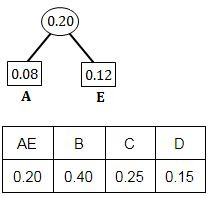Step 2: Select two characters from Step 1 with smallest probabilities and merge them.Step 3: Select two characters (from Step 2) with smallest probabilities and merge them.Step 4: Merge the remaining two probabilities.A = 1000 (4-bits)
E = 1001 (4-bits)
D = 101 (3-bits)
C = 11 (2-bits)
B = 0 (1-bit)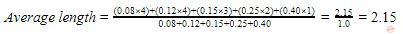Question 34

A binary search tree in which every non-leaf node has non-empty left and right subtrees is called a strictly binary tree. Such a tree with 19 leaves :

 A cannot have more than 37 nodes B has exactly 37 nodes C has exactly 35 nodes D cannot have more than 35 nodes
Algorithms       Binary-search-tree       UGC-NET JUNE Paper-2
Question 34 Explanation:
L = I(n - 1)+ 1
Where,
L = Number of leaf nodes
n = N-ary tree
I = Number of intermediate nodes
Now,
19 = I(2 - 1) + 1
19 = 2I - I + 1
19 = I + 1
I = 18
Total Number of nodes in a tree = Number of Intermediate nodes + Number of Leaf nodes
Total Number of nodes in a tree = 19 + 18
Total Number of nodes in a tree = 37
 Question 35

Match the following with respect to algorithm paradigms :

```          List-I                        List-II
(a) The 8-Queens problem               (i) Dynamic programming
(b) Single-Source shortest paths      (ii) Divide and conquer
(c) STRASSEN’s Matrix multiplication (iii) Greedy approach
(d) Optimal binary search trees       (iv) Backtracking

```

 A (a)-(iv), (b)-(i), (c)-(iii), (d)-(ii) B (a)-(iv), (b)-(iii), (c)-(i), (d)-(ii) C (a)-(iii), (b)-(iv), (c)-(ii), (d)-(i) D (a)-(iv), (b)-(iii), (c)-(ii), (d)-(i)
Question 35 Explanation:
8-Queens problem use Backtracking.
Single-Source shortest paths use Greedy approach.
STRASSEN’s Matrix multiplication use Divide and conquer technique.
Optimal binary search trees use Dynamic programming.
 Question 36
The maximum number of comparisons needed to sort 9 items using radix sort is (assume each item is 5 digit octal number) :
 A 45 B 72 C 360 D 450
Algorithms       Sorting       UGC-NET JUNE Paper-2
Question 36 Explanation:
Total sort items = 9
Octal number having → 5 digits
The octal number system base value = 8
The maximum number of comparison = (number of items) * (radix) * (number of digits)
= 9 * 5 * 8
= 360
 Question 37

A 5-ary tree is tree in which every internal node has exactly 5 children. The number of left nodes in such a tree with 8 internal nodes will be :

 A 30 B 33 C 45 D None of the above
Algorithms       Binary-search-tree       UGC-NET JUNE Paper-2
Question 37 Explanation:
**They excluded for Evaluation** . We given how to approach this problem.
L = I(n - 1) + 1
Where,
L = Number of leaf nodes
n = N-ary tree
I = Number of intermediate nodes
Now, L = 8(5 - 1) + 1
L = 8(4) + 1
L = 33
Total Number of nodes in a tree = Number of Intermediate nodes + Number of Leaf nodes
Total Number of nodes in a tree = 8 + 33
Total Number of nodes in a tree = 41
 Question 38

Consider a Boolean function of ‘n’ variables. The order of an algorithm that determines whether the Boolean function produces a output 1 is :

 A Logarithmic B Linear C Quadratic D Exponential
Algorithms       Boolean-functions       UGC-NET JUNE Paper-2
Question 38 Explanation:Set 1 have “n” variables and each variable in set 1 can be mapped with one boolean value in set 2 i.e,. each variable in set 1 have 2 choices and we have “n” such variables in set 1.
→ So, total number of choices we get maximum 2n. It means exponential.
 Question 39

E is the number of edges in the graph and f is maximum flow in the graph. When the capacities are integers, the runtime of Ford-Fulkerson algorithm is bounded by :

 A O(E∗f) B O(E2∗f) C O(E∗f2) D None of the above
Algorithms       Greedy-approach       UGC-NET JUNE Paper-2
Question 39 Explanation:
****Excluded for evaluation***
→ The Ford-Fulkerson method or Ford-Fulkerson algorithm (FFA) is a greedy algorithm that computes the maximum flow in a flow network.
→ It is called a "method" instead of an "algorithm" as the approach to finding augmenting paths in a residual graph is not fully specified or it is specified in several implementations with different running times.
→ the runtime of Ford-Fulkerson is bounded by O(E*f), where E is the number of edges in the graph and f is the maximum flow in the graph. This is because each augmenting path can be found in O(E) time and increases the flow by an integer amount of at least 1, with the upper bound f.
→ The variation of the Ford-Fulkerson algorithm with guaranteed termination and a runtime independent of the maximum flow value is the Edmonds-Karp algorithm, which runs in O(VE2)time.
 Question 40
For the given recurrence equation
T(n)=2T(n-1), if n>0.
=1, otherwise
 A O(nlogn) B O(n2) C O(2n) D O(n)
Algorithms       Nielit Scentist-B [02-12-2018]
Question 40 Explanation:
T(n) = 2T (n - 1) + 1
= 2 [2T(n - 2) + 1] + 1
= 22 T(n - 2) + 3

= 2kT(n - k) + (2k - 1)
= 2n-1T(1) + (2n-1 - 1)
= 2n-1+ 2n-1 - 1
= 2n - 1
≅ O( 2n )
 Question 41
The smallest element that can be found in time___ in a binary max heap
 A O(nlogn) B O(logn) C O(n) D O(n2)
Algorithms       Nielit Scentist-B [02-12-2018]
Question 41 Explanation:
We need to traverse all nodes in order to identify smallest element because it is not following binary search tree properties. Searching time for ‘n’ elements will take O(n) in worst case.
 Question 42
___ is the worst case time complexity for all operations(i.e. Search,update and delete) in a general binary search tree
 A O(n) B O(nlogn) C O(logn) for search and insert, and O(n) for delete D None of these
Algorithms       Nielit Scentist-B [02-12-2018]
Question 42 Explanation:
→ Suppose the elements are not in sorted order, it will take O(n) time for worst case time complexity.
→ In question, they are not mentioned about elements are sorted or unsorted. So, worst case we have to consider unsorted elements.
→ All operations(i.e. Search,update and delete) will take O(n).
 Question 43
Dijkstra’s algorithm is based on:
 A Greedy approach B Dynamic programming C Backtracking paradigm D Divide and conquer paradigm
Algorithms       Nielit Scentist-B [02-12-2018]
Question 43 Explanation:
→ Dijkstra's algorithm is following greedy approach. It always selects shortest path among all possibilities.
→ Dijkstra’s algorithm is solving the problem of single source shortest path.
 Question 44
For the given nodes:
89,19,50,17,12,15,2,5,7,11,6,9,100
Minimum _____ number of interchanges are needed to convert it into a max-heap
 A 3 B 4 C 5 D 6
Algorithms       Nielit Scentist-B [02-12-2018]
Question 44 Explanation:
1st swap is: 100 and 15
2nd swap is: 100 and 50
3rd swap is: 100 and 89Question 45
_____sorting algorithms has the lowest worst case complexity
 A Selection sort B Bubble sort C Merge sort D Quick sort
Algorithms       Nielit Scentist-B [02-12-2018]
Question 45 Explanation:
The Worst case time complexity of Selection,Bubble and Quick sort is O(n2) where as Merge sort is O(nlog(n)). So Merger sort has lowest worst case time complexity
 Question 46
Two alternative packages A and B are available for processing a database having 10k records. Package A requires 0.0001n​2​ time units and package B requires 10nlog​10​n time units​ ​ to process n records. What is the smallest value of k for which package B will be preferred over A?
 A 12 B 10 C 6 D 5
Algorithms       Asymptotic-Complexity       Nielit Scientist-B IT 4-12-2016
Question 46 Explanation:
As per given information Package B 10nlog​ 10​ n is lesser than or equals to Package A 0.0001n​ 2​ 0
because n​ 2​ is asymptotically larger than nlogn. Finally, 10nlog​ 10​ n ≤ 0.0001n​ 2
Let n = 10​ k​ records. Substitute into 10nlog​ 10​ n ≤ 0.0001n​ 2
10(10​ k​ )log​ 10​ 10​ k​ ≤ 0.0001(10​ k​ )​ 2
10​ k+1​ k ≤ 0.0001 × 10​ 2k
k ≤ 10​ 2k−k−1−4
k ≤ 10​ k−5
According to the problem value 6 is suitable for K.
 Question 47
What is the type of the algorithm used in solving the 4 Queens problem?
 A Greedy B Branch and bound C Dynamic Programming D Backtracking
Algorithms       Algorithm-Paradigms       Nielit Scientist-B IT 4-12-2016
Question 47 Explanation:
N-Queen problem:​ an arrangement of N queens on a chess board, such that no queen can attack any other queens on the board.The chess queens can attack in any direction as horizontal, vertical, horizontal and diagonal way. A binary matrix is used to display the positions of N Queens, where no queens can attack other queens.
Backtracking is a general algorithm for finding all (or some) solutions to some computational problems, notably constraint satisfaction problems, that incrementally builds candidates to the solutions, and abandons a candidate ("backtracks") as soon as it determines that the candidate cannot possibly be completed to a valid solution.
 Question 48
selection sort,quick sort is a stable sorting method
 A True,True B False, False C True,False D False,False
Algorithms       Sorting       Nielit Scientist-B IT 4-12-2016
Question 48 Explanation: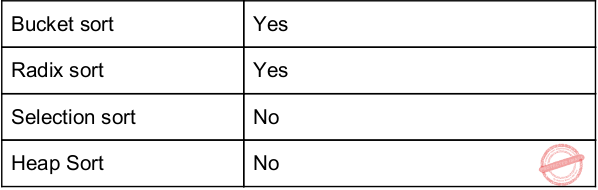Question 49
Which of the following sorting procedures is the slowest?
 A Quick sort B Merge sort C Shell sort D Bubble sort
Algorithms       Sorting       Nielit Scientist-B IT 4-12-2016
Question 49 Explanation:
Bubble sort will execute O(n​ 2​ ) time worst case and also it takes n-1 comparisons. So, bubble sort procedure is the slowest one among all.
 Question 50
The recurrence relation capturing the optimal execution time of the towers of Hanoi problem with n discs is:
 A T(n)=2T(n-2)+2 B T(n)=2T(n/2)+1 C T(n)=2T(n-2)+n D T(n)=2T(n-1)+1
Algorithms       Recurrences       Nielit Scientist-B IT 4-12-2016
Question 50 Explanation:
The recurrence equation for given recurrence function is
T(n) = 2T(n – 1) + 1
= 2 [2T(n – 2) + 1] + 1
= 2​ 2​ T(n – 2) + 3

= 2​ k​ T( n – k) + (2​ k​ – 1)
n – k = 1
= 2​ n-1​ T(1) + (2​ n-1​ – 1)
= 2​ n-1​ + 2​ n-1​ – 1
= 2​ n​ – 1
≌ O(2​ n​ )
 Question 51
Complexity of kruskal's algorithm for finding minimum spanning tree of an undirected graph containing n vertices and m edges if the edges are sorted is:
 A O(mn) B O(m) C O(m+n) D O(n)
Algorithms       Asymptotic-Complexity       Nielit Scientist-B IT 4-12-2016
Question 51 Explanation:
Implementation of Kruskal's algorithm should be implemented in 2 steps:
Step1: Sorting of edges takes O(ELogE) time.
Step2: After sorting, we iterate through all edges and apply find union algorithm.
The find and union operations can take at most O(1) time if you use Disjoint set . So overall complexity is O(ELogE + E) time.
Given the edges are already sorted, so we need to do only second step i.e.,we iterate through all edges and apply find-union algorithm. The find and union operations can take at most O(1) time. So, the total time complexity will be O(E).
 Question 52
Two alternative package A and B are available for processing a database having 10​ k records. Package A requires 0.00012n​​ time units and package B requires 10nlog​​10 n time units to process n records. What is the smallest value of k for which package B will be preferred over A?
 A 12 B 10 C 6 D 5
Algorithms       Time-Complexity       Nielit Scientist-C 2016 march
Question 52 Explanation:
As per given information Package B 10nlog​ 10​ n is lesser than or equals to Package A 0.0001n​ 2​0
because n​2​ is asymptotically larger than nlogn. Finally, 10nlog​ 10​ n ≤ 0.0001n​ 2
Let n = 10​ k​ records. Substitute into 10nlog​ 10​ n ≤ 0.0001n​ 2
10(10​ k​ )log​ 10​ 10k​ ≤ 0.0001(10​ k​ )​ 2
10​ k+1​ k ≤ 0.0001 × 10​ 2k
k ≤ 10​
2k−k−1−4
k ≤ 10
k−5
According to the problem value 6 is suitable for K.
 Question 53
You are given the postorder traversal,p, of a binary search tree on the n elements 1,2,..,n. You have to determine the unique binary search tree has P as its postorder traversal. What is the time complexity of the most efficient algorithm for doing this?
 A O(logn) B O(n) C O(nlogn) D none of the above, as the tree cannot be ​ be uniquely determined.
Algorithms       Asymptotic-Complexity       Nielit Scientist-C 2016 march
Question 53 Explanation:
Last element in post order is the root of tree- find this element in inorder-log n time. Now as in quick sort consider this as pivot and split the post order array into 2. All elements smaller than pivot goes to left and all elements larger than pivot goes to right and suppose we have k elements smaller than pivot, these elements will be same in both in-order as well as post order. Now, we already got the root, now left child is the left split and right child is the right split.
T(n) = T(k) + T(n-k-1) + logn
In worst case T(n) = O(nlogn), when k=0
But searching for an element in the in-order traversal of given BST can be done in O(1) because we have n elements from 1...n so there is no need to search an element- if last element in post order is say 5 we take it as root and since 4 elements are split the post order array into two (first 4 elements), (6th element onward) and solve recursively. Time complexity for this would be
T(n) = T(k) + T(n-k-1)+O(1)
Which gives T(n) = O(n)
since we know that all elements must be traversed at least once T(n) = θ(n)
 Question 54
The most efficient algorithm for finding the number of connected components in a n undirected graph on n vertices and m edges has time complexity
 A O(n) B O(m) C O(m+n) D O(mn)
Algorithms       Asymptotic-Complexity       Nielit Scientist-C 2016 march
Question 54 Explanation:
To find the number of connected components using either BFS or DFS time complexity is θ(m+n).
Suppose if we are using Adjacency matrix means it takes θ(n​ 2​ ).
 Question 55
An element in an array X is called a leader if it is greater than all elements to the right of it in X. The best algorithm to find all leaders in an array
 A solves it in linear time using a left to right pass of the array B solves in linear time using a right to left pass of the array C solves it using divide and conquer in time O(nlogn) D solves it in time O(n​ 2​ )
Algorithms       Time-Complexity       Nielit Scientist-C 2016 march
Question 55 Explanation:
Algorithm to solve the problem :
● Start from the right keeping track of largest element (currentLeader). If a larger element is found, then print it and set currentLeader to the current element.
● We can consider the last element is a leader since there is no element to its right. Solves it in linear time using a right to left pass of the array will takes time complexity is O(n).
 Question 56

Consider the following terminology and match List 1 and List 2 and choose the correct answer from the code given below

b = branch factor
d = depth of shallowest solution
M = Maximum depth of the search tree
l = depth limit
```    List 1                          List 2
(a) BFS                          i) O(bd)
(b) DFS                         ii) O(bd)
(c) Depth- Limited Search      iii) O(bm)
(d) Iterative deepening Search  iv) O(bl)```
Code:
 A (a)-(iii), (b)-(ii), (c)-(iv), (d)-(i) B (a)-(ii), (b)-(iii), (c)-(iv), (d)-(i) C (a)-(i), (b)-(ii), (c)-(iv), (d)-(iii) D (a)-(i), (b)-(iii), (c)-(iv), (d)-(ii)
Algorithms       Greedy-approach       UGC-NET DEC Paper-2
Question 56 Explanation:
BFS → O(bd) worst case space complexity
DFS → O(bm) worst case space complexity
Depth - Limited Search → O(bl)
Iterative deepening Search → O(bd)
Note:
Based upon BFS and DFS we can find the solution.
 Question 57

Match List-1 with List-2 and choose the correct answer from the code given below

```           List 1					   List 2
(a) Greedy Best-first Search         	 (i) Selects a node for expansion if optimal path to
that node has been found
(b) A* Search	          		(ii) Avoids substantial overhead associated with
keeping the sorted queue of nodes
(c) Recursive Best-First Search        (iii) Suffers from excessive node generation
(d) Iterative-deepening A* Search   	(iv) Time complexity depends upon the quality of
heuristic```
Code:
 A (a)-(iv), (b)-(iii), (c)-(ii), (d)-(i) B (a)-(i), (b)-(iv), (c)-(iii), (d)-(ii) C (a)-(iv), (b)-(i), (c)-(ii), (d)-(iii) D (a)-(i), (b)-(ii), (c)-(iii), (d)-(iv)
Algorithms       Match-the-following       UGC-NET DEC Paper-2
Question 57 Explanation:
Greedy Best-first Search: Selects a node for expansion if optimal path to that node has been found. Best-first search is a search algorithm which explores a graph by expanding the most promising node chosen according to a specified rule. Best-first search as estimating the promise of node n by a "heuristic evaluation function f(n) which, in general, may depend on the description of n, the description of the goal, the information gathered by the search up to that point, and most important, on any extra knowledge about the problem domain."
A* Search: Time complexity depends upon the quality of heuristic.
Recursive Best-First Search: Suffers from excessive node generation. RBFS depends on how widely the promising nodes are separated in the search tree, and is harder to anticipate.
Iterative-deepening A* Search: Avoids substantial overhead associated with keeping the sorted queue of nodes.
 Question 58

Consider the graph shown below :Use Kruskal’s algorithm to find the minimum spanning tree of the graph. The weight of this minimum spanning tree is

 A 13 B 16 C 17 D 14
Algorithms       Minimum-spanning-Tree       UGC-NET DEC Paper-2
Question 58 Explanation:The weight of this minimum spanning tree is 16.
 Question 59

Match List 1 with List 2 and choose the correct answer from the code given below :

```       List I                           List II
(Graph Algorithm)               (Time Complexity)
(a) Dijkstra’s algorithm            (i) O(E lg E)
(b) Kruskal’s algorithm            (ii) Θ(V3)
(c) Floyd-Warshall algorithm      (iii) O(V2)
(d) Topological sorting            (iv) Θ(V + E)```

Where V and E are the number of vertices and edges in graph respectively.

Code :
 A (a)-(i), (b)-(iii), (c)-(iv), (d)-(ii) B (a)-(i), (b)-(iii), (c)-(ii), (d)-(iv) C (a)-(iii), (b)-(i), (c)-(ii), (d)-(iv) D (a)-(iii), (b)-(i), (c)-(iv), (d)-(ii)
Algorithms       Time-Complexity       UGC-NET DEC Paper-2
Question 59 Explanation:
→ Dijkstra's algorithm (or Dijkstra's Shortest Path First algorithm, SPF algorithm) is an algorithm for finding the shortest paths between nodes in a graph and time complexity is O(V2).
→ Kruskal's algorithm is a minimum-spanning-tree algorithm which finds an edge of the least possible weight that connects any two trees in the forest and time complexity is O(E log E).
→ A topological sort or topological ordering of a directed graph is a linear ordering of its vertices such that for every directed edge uv from vertex u to vertex v,u comes before v in the ordering Θ(V + E).
→ Floyd–Warshall algorithm is an algorithm for finding shortest paths in a weighted graph with positive or negative edge weights. A single execution of the algorithm will find the lengths of shortest paths between all pairs of vertices.
 Question 60

The second smallest of n elements can be found with _______ comparisons in worst case.

 A n + ceil(lg n) - 2 B n - 1 C lg n D 3n/1
Algorithms       Time-Complexity       UGC-NET DEC Paper-2
Question 60 Explanation:This takes n-1 comparisons and each element involved is compared at most log n times. Finally, we get the largest element A1’’’. This element which is largest, has won comparison with the second largest element at some point. So, keeping track of items compared with the largest element will give us a list of (log n) elements. In this list we find out largest, which will be the second largest of all.
So, no. of comparisons = (n - 1) + log2 (n) - 1
Example: 512 elements
In this case no. of comparisons will be, (512 - 1) + log2(512) - 1
= 511 + (9 - 1)
= 511 + 8
= 519
 Question 61

Consider two sequences X and Y :

X = <0,1,2,1,3,0,1 >
Y = <1,3,2,0,1,0 >

The length of longest common subsequence between X and Y is

 A 4 B 2 C 3 D 5Computer-Networks
Algorithms       Longest-common-subsequesnce       UGC-NET DEC Paper-2
Question 61 Explanation:Possible subsequences are : 130, 1210, 1301 and length of longest common subsequence is 4.
 Question 62

The solution of recurrence relation : T(n) = 2T(sqrt(n)) + lg(n) is

 A O(lg (n) lg(n)) B O(lg (n)) C O(n lg (n)) D O(lg (n) lg(lg(n)))
Algorithms       Recurrence-Relations       UGC-NET DEC Paper-2
Question 62 Explanation: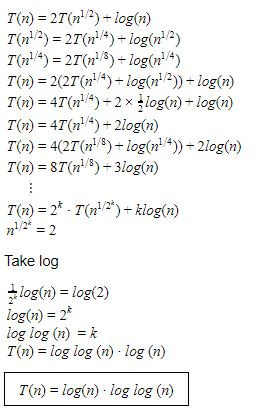Question 63
The worst case running times of insertion sort, merge sort and quick sort respectively are
 A O(nlogn),O(nlogn) and O(n2) B O(n2),O(n2) and O(nlogn) C O(n2),O(nlogn) and O(nlogn) D O(n2),O(nlogn) and O(n2)
Algorithms       Sorting       Nielit Scientist-B CS 22-07-2017
Question 63 Explanation:
→ worst case running times of insertion sort → O(n2)
merge sort → O(nlogn)
quick sort → O(n2)
 Question 64
Which of the following standard algorithms is not Dynamic Programming based?
 A Bellman ford algorithm for single source shortest path B floyd Warshall for all pairs shortest paths C 0-1 knapsack problem D Prim’s minimum spanning tree
Algorithms       Dynamic-Programming       Nielit Scientist-B CS 22-07-2017
Question 64 Explanation:
Prim’s minimum spanning tree is following greedy technique.
 Question 65
Kadane algorithm is used to find
 A Maximum sum subsequence in an array B Maximum sum subarray in an array C Maximum product subsequence in an array D Maximum product subarray in an array
Algorithms       Dynamic-Programming       Nielit Scientist-B CS 22-07-2017
Question 65 Explanation:
Kadane algorithm is used to find the maximum sum subarray in an array. It runs in O(n) time complexity Implementation in python:
def max_subarray(A):
max_ending_here = max_so_far = A
for x in A[1:]:
max_ending_here = max(x, max_ending_here + x)
max_so_far = max(max_so_far, max_ending_here)
return max_so_far
 Question 66
Given an random unsorted array ‘A’ in which every element is at most ‘d’ distance from is position in sorted array where d<Size(A). If we applied the insertion sort over this array, then the time complexity of algorithm is:
 A O(nlogd) B O(n2 logd) C O(nd) D O(n2d)
Algorithms       Nielit STA [02-12-2018]
Question 66 Explanation:
→ Using insertion sort worst case time complexity is O(n2).
→ They are asking to find the atmost distance from every element. So, it will take O(n2*d) from every node.
Note: Using heap sort , time complexity will be O(nlogd) and most effective than insertion sort.
 Question 67
If G be an undirected connected graphs with distinct edge weight such that maximum weight and minimum weight of edge is given by emax and emin respectively. Then, Which of the following statements is TRUE?
 A Every minimum spanning tree of G must contain emin and emax B If emax is in a minimum spanning tree, then its removal must disconnect G C No minimum spanning tree contains emax D G has a multiple minimum spanning trees
Algorithms       Nielit STA [02-12-2018]
Question 67 Explanation:Question 68
Consider bottom up merge sort working on ‘n’ elements such that n=2i. The minimum number of comparisons in order to get sorted list is:
 A nlog(n/2) B nlogn C (nlogn)/2 D logn
Algorithms       Nielit STA [02-12-2018]
Question 68 Explanation:
Minimum comparison is only possible when first and last element of either array are smaller then first element of another. so in such as case at each level we will have n/2 comparisons In general= n/2 * number of levels = (nlogn)/2Question 69
The time complexity of the Tarjan’s algorithm for finding the strongly connected components of a graph having m vertices and n edges is:
 A O(m*n) B O(m+n) C O(m2) D O(mn)
Algorithms       Nielit STA [02-12-2018]
Question 69 Explanation:
Tarjan Algorithm
1. DFS search produces a DFS tree/forest
2. Strongly Connected Components form subtrees of the DFS tree.
3. If we can find head of such subtrees, we can print/store all the nodes in that subtree (including head) and that will be one SCC.
4. There is no back edge from one SCC to another (There can be cross edges, but cross edges will not be used while processing the graph).
Note: Time complexity for Tarjan’s algorithm is O(V+E)

Kosaraju’s algorithm
1) Create an empty stack ‘S’ and do DFS traversal of a graph. In DFS traversal, after calling recursive DFS for adjacent vertices of a vertex, push the vertex to stack. In the above graph, if we start DFS from vertex 0, we get vertices in stack as 1, 2, 4, 3, 0.
2) Reverse directions of all arcs to obtain the transpose graph.
3) One by one pop a vertex from S while S is not empty. Let the popped vertex be ‘v’. Take v as source and do DFS (call DFSUtil(v)). The DFS starting from v prints strongly connected component of v. In the above example, we process vertices in order 0, 3, 4, 2, 1 (One by one popped from stack).
Note: Time complexity for kosaraju’s algorithm is O(V+E)
 Question 70
which of the following sorting algorithms does not have a worst case running time of O(n​ 2​ )
 A Insertion sort B merge sort C Quick sort D bubble sort
Algorithms       Sorting       Nielit Scientist-B CS 2016 march
Question 70 Explanation:Question 71
If there is in NP-Complete language L whose complement is in NP, then complement of any language in NP is in
 A P B NP C Both (A) and (B) D None of these
Algorithms       NP complete       Nielit Scientist-B CS 2016 march
Question 71 Explanation:
If there is in NP-Complete language L whose complement is in NP, then complement of any language in NP is in NP
 Question 72
Time complexity of an algorithm T(n), where n is the input size is given by T(n)=T(n-1)+1/n, if n>1 =1, otherwise The order of this algorithm is
 A logn B n C n​ 2 D n​ n
Algorithms       Recurrences       Nielit Scientist-B CS 2016 march
Question 72 Explanation:
T (n) = T (n − 1 ) + 1/nQuestion 73
To sort many large object or structures, it would be most efficient to
 A Place reference to them in and array an sort the array B place them in a linked list and sort the linked list C place pointers to them in an array and sort the array D place them in an array and sort the array
Algorithms       Sorting       NieLit STA 2016 March 2016
Question 73 Explanation:
Option-A is suitable for small amount of objects but not large
Option-B is not correct because it will extra time complexity and searching very difficult
Option-D is suitable for small amount of objects/elements
 Question 74
Consider the following terminology and match List 1 and List 2 and choose the correct answer from the code given below
b= branch factor
d= depth of shallowest solution
M= Maximum depth of the search tree
I= depth limitA (a)-(iii), (b)-(ii), (c)-(iv), (d)-(i) B (a)-(ii), (b)-(iii), (c)-(iv), (d)-(i) C (a)-(i), (b)-(ii), (c)-(iv), (d)-(iii) D (a)-(i), (b)-(iii), (c)-(iv), (d)-(ii)
Algorithms       Time-Complexity       UGC NET CS 2018-DEC Paper-2
Question 74 Explanation:
BFS → O( b d ) worst case space complexity
DFS → O(bm) worst case space complexity
Depth- Limited Search → O(bl)
Iterative deepening Search → O(bd)
Note: Based upon BFS and DFS we can find the solution.
 Question 75
Match List 1 with List 2 and choose the correct answer from the code given belowA (a)-(iv), (b)-(iii), (c) -(ii), (d)-(i) B (a)-(i), (b)-(iv), (c) -(iii), (d)-(ii) C (a)-(iv), (b)-(i), (c) -(ii), (d)-(iii) D (a)-(i), (b)-(ii), (c) -(iii), (d)-(iv)
Algorithms       Match-The-Following       UGC NET CS 2018-DEC Paper-2
Question 75 Explanation:
→ ​ Greedy Best-first Search:​ Selects a node for expansion if optimal path to that node has been found. Best-first search is a search algorithm which explores a graph by expanding the most promising node chosen according to a specified rule. Best-first search as estimating the promise of node n by a "heuristic evaluation function f(n) which, in general, may depend on the description of n, the description of the goal, the information gathered by the search up to that point, and most important, on any extra knowledge about the problem domain."
→ ​ A* Search:​ Time complexity depends upon the quality of heuristic.
→ ​ Recursive Best-First Search:​ Suffers from excessive node generation. RBFS depends on how widely the promising nodes are separated in the search tree, and is harder to anticipate.
→ ​ Iterative-deepening A* Search:​ Avoids substantial overhead associated with keeping the sorted queue of nodes
 Question 76
In a ternary tree, the number of internal nodes of degree 1,2 and 3 is 4,3 and 3 respectively. The number of leaf nodes in the ternary tree is
 A 11 B 12 C 10 D 9
Algorithms       Trees       UGC NET CS 2018-DEC Paper-2
Question 76 Explanation:
Number of internal nodes= 4+3+3= 10
Number of leaf nodes = Sum of degrees of all internal nodes - number of internal nodes +1
= (4*1 + 3*2 + 3*3 )-10 +1
= 19- 10 +1=10
Formula​ : nd-n +1 where ​ n ​ is number of nodes and d ​ is degree of the tree.
 Question 77
Consider the graph shown below :Use Kruskal’s algorithm to find the minimum spanning tree of the graph. The weight of this minimum spanning tree is
 A 13 B 16 C 17 D 14
Algorithms       Graphs       UGC NET CS 2018-DEC Paper-2
Question 77 Explanation: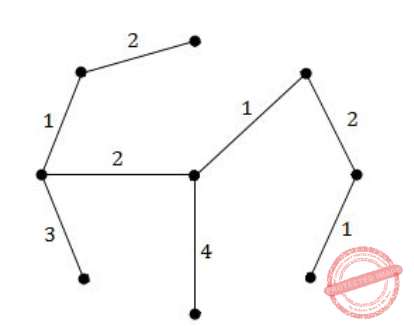The weight of this minimum spanning tree is 16.
 Question 78
The second smallest of n elements can be found with _______ comparisons in worst case.
 A n + ceil(lg n) -2 B n-1 C lg n D 3n/1
Algorithms       Time-Complexity       UGC NET CS 2018-DEC Paper-2
Question 78 Explanation:This takes n-1 comparisons and each element involved is compared at most log n times. Finally, we get the largest element A1’’’ . This element which is largest, has won comparison with the second largest element at some point. So, keeping track of items compared with the largest element will give us a list of (log n) elements. In this list we find out largest, which will be the second largest of all.
So, no. of comparisons =(n-1)+log​ 2​ (n)-1
Example: 512 elements
In this case no. of comparisons will be, (512-1)+log​ 2​ (512) -1
=511+(9-1)
=511+8
=519
 Question 79
Consider two sequences X and Y :
X = <0,1,2,1,3,0,1 >
Y = <1,3,2,0,1,0 >
The length of longest common subsequence between X and Y is
 A 4 B 2 C 3 D 5
Algorithms       LCS       UGC NET CS 2018-DEC Paper-2
Question 79 Explanation:Possible subsequences are :130, 1210, 1301 and length of longest common subsequence is 4.
 Question 80
Consider the midpoint (or Bresenham) algorithm for rasterizing lines given below :
(1) Input (x​ 1​ ,y​ 1​ ) and (x​ 2​ ,y​ 2​ )
(2) y=y​ 1
(3) d=f(x​ 1​ +1, y​ 1​ +1⁄2) // f is the implicit form of a line
(4) for x=x​ 1​ to x​ 2
(5) do
(6) plot(x,y)
(7) if(d<0)
(8) then
(9) y=y+1
(10) d=d+(y​ 1​ - y​ 2​ ) + (x​ 2​ - x​ 1​ )
(11) else
(12) d=d+(y​ 1​ - y​ 2​ )
(13) end
(14) end
Which statements are true ?
P: For a line with slope m>1, we should change the outer loop in line (4) to be over y.
Q: Lines (10) and (12) update the decision variable d through an incremental evaluation of the line equation f.
R: The algorithm fails if d is ever 0.
 A Q and R only B P only C P and Q only D P,Q and R
Algorithms       Bresenham       UGC NET CS 2018-DEC Paper-2
Question 80 Explanation:
From the code given in question gives information that the algorithm will work if d is ever 0, So the statement R is false.
Line 10 and 12 will update the value of d , So the statement Q is true.
 Question 81
In PERT/CPM, the merge event represents___________ of two or more events.
 A splitting B completion C beginning D joining
Algorithms       PERT/CPM        UGC NET CS 2018-DEC Paper-2
Question 81 Explanation:
→ The program (or project) evaluation and review technique (PERT) is a statistical tool used in project management, which was designed to analyze and represent the tasks involved in completing a given project.
→ The critical path method (CPM), or critical path analysis (CPA), is an algorithm for scheduling a set of project activities. It is commonly used in conjunction with the program evaluation and review technique (PERT).
→ A critical path is determined by identifying the longest stretch of dependent activities and measuring the time required to complete them from start to finish.
→ ​ Event: An event represents a point in time signifying the completion of some activities and the beginning of new ones. This is usually represented by a circle in a network which is also called a node or connector. The events are classified into three categories
1. Merge event – When more than one activity comes and joins an event such an event is known as merge event.
2. Burst event – When more than one activity leaves an event such an event is known as burst event.
3. Merge and Burst event – An activity may be merge and burst event at the same time as with respect to some activities it can be a merge event and with respect to some other activities it may be a burst event.Question 82
​The solution of recurrence relation : T(n)=2T(sqrt(n))+lg(n) is
 A O(lg (n) lg(n)) B O(lg (n)) C O(n lg (n)) D O(lg (n) lg(lg(n)))
Algorithms       Recurrences       UGC NET CS 2018-DEC Paper-2
Question 82 Explanation:T (n) = l og log (n) · l og (n)
T (n) = l og(n) · l og log (n)
 Question 83
The Knapsack problem belongs to which domain of problems?
 A Optimization B NP complete C Linear Solution D Sorting
Algorithms       0/1-Knapsack-and-fractional-knapsack       Nielit Scientist-B CS 4-12-2016
Question 83 Explanation:
The knapsack problem or rucksack problem is a problem in combinatorial optimization: Given a set of items, each with a weight and a value, determine the number of each item to include in a collection so that the total weight is less than or equal to a given limit and the total value is as large as possible
 Question 84
The running time of quick sort algorithm depends heavily on the selection of:
 A No. of inputs B Arrangement of elements in an array C Size of elements D Pivot element
Algorithms       Sorting       Nielit Scientist-B CS 4-12-2016
Question 84 Explanation:
The running time of Quicksort will depend on how balanced the partitions are. If you are unlucky and select the greatest or the smallest element as the pivot, then each partition will separate only one element at a time, so the running time will be similar to Insertion Sort.However, Quicksort will usually pick a pivot that is mid-range, and it will partition the array into two parts.
 Question 85
Two main measures for the efficiency of an algorithm are:
 A Processor and Memory B Complexity and Capacity C Time and Space D Data and Space
Algorithms       Asymptotic-Complexity       Nielit Scientist-B CS 4-12-2016
Question 85 Explanation:
The complexity of an algorithm is a function describing the efficiency of the algorithm in terms of the amount of data the algorithm must process. Usually there are natural units for the domain and range of this function. There are two main complexity measures of the efficiency of an algorithm:
Time complexity is a function describing the amount of time an algorithm takes in terms of the amount of input to the algorithm. "Time" can mean the number of memory accesses performed, the number of comparisons between integers, the number of times some inner loop is executed, or some other natural unit related to the amount of real time the algorithm will take. We try to keep this idea of time separate from "wall clock" time, since many factors unrelated to the algorithm itself can affect the real time (like the language used, type of computing hardware, proficiency of the programmer, optimization in the compiler, etc.). It turns out that, if we chose the units wisely, all of the other stuff doesn't matter and we can get an independent measure of the efficiency of the algorithm.
Space complexity is a function describing the amount of memory (space) an algorithm takes in terms of the amount of input to the algorithm. We often speak of "extra" memory needed, not counting the memory needed to store the input itself. Again, we use natural (but fixed-length) units to measure this. We can use bytes, but it's easier to use, say, number of integers used, number of fixed-sized structures, etc. Space complexity is sometimes ignored because the space used is minimal and/or obvious, but sometimes it becomes as important an issue as time.
 Question 86
What is the solution to the recurrence T(n)=T(n/2)+n?
 A O(log n) B O(n) C O(n logn) D None of these
Algorithms       Asymptotic-Complexity       Nielit Scientist-B CS 4-12-2016
Question 86 Explanation:
The above recurrence is in the form of masters theorem.
a=1,b=2,k=1,p=0
=ak
=O(n)
 Question 87
The concept of order Big O is important because:
 A It can be used to decide the best algorithm that solves a given problem B It is the lower bound of the growth rate of algorithm C It determines the maximum size of a problem that can be solved in a given amount of time D Both (A) and (B)
Algorithms       Asymptotic-Complexity       Nielit Scientist-B CS 4-12-2016
Question 87 Explanation:
● Big O notation is used to classify algorithms according to how their running time or space requirements grow as the input size grows.
● The letter O is used because the growth rate of a function is also referred to as the order of the function.
● A description of a function in terms of big O notation usually only provides an upper bound on the growth rate of the function.
 Question 88
0/1-Knapsack is a well known problem where, it is desired to get the maximum total profit by placing n items(each item is having some weight and associated profit) into a knapsack of capacity W. The table given below shows the weights and associated profits for 5 items, where one unit of each item is available to you. It is also given that the knapsack capacity W is 8. If the given 0/1 knapsack problem is solved using Dynamic Programming, which one of the following will be maximum earned profit by placing the items into the knapsack of capacity 8.A 19 B 18 C 17 D 20
Algorithms       0/1-Knapsack-and-fractional-knapsack       Nielit Scientist-B IT 22-07-2017
Question 88 Explanation:
Here, we can get the first item using profit per weight even we are using 0/1 knapsack.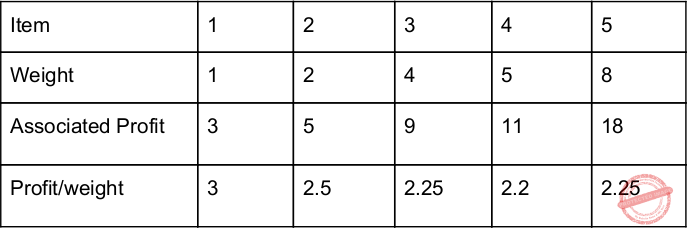→ According to above Profit/weight ratio, the item-1 having maximum profit. So, pick item-1.
→ Now M=7, Pick item-2 because it has more profit.
→ Now M=5, Now according to profit/weight ration we can pick item-3 or item-5 but item-5 having more weight, so it exceeds capacity. If we are selecting item-3 means we are wasting 1 slot. So, here, profit/weight ration fails. So, follow brute force technique in this position. We can pick item-4 because it has weight 5.
→ Now, M=0 and Profit=19. [item1 + item2 + item4]
 Question 89
What is the running time of the following function(specified as a function of the input value)?
void Function(int n)
{
int i=1;
int s=1;
while(s<=n)
{
i++;
s=s+i;
}
}
 A O(n) B O(n​ 2​ ) C O(1) D O(​√n)
Algorithms       Asymptotic-Complexity       Nielit Scientist-B IT 22-07-2017
Question 89 Explanation:
S= 1, 3, 6, 10, 15 21….(>n) stopping condition.
i= 1, 2, 3, 4, 5, 6…..
Here, ‘S’ is nothing but sum of ‘n’ natural numbers
Assume the n=21, then corresponding k value 6. "k^2" is nothing but 36 which is greater than "n"
For any given K value , the S value is k(k+1)/2, So, 6(6+1)/2=21
the loop will terminate whenever k(k+1)/2 >n
k=O(√n)
 Question 90
A machine needs a minimum of 100 sec to sort 1000 names by quick sort. The minimum time needed to sort 100 names will be approximately
 A 50.2 sec B 6.7 sec C 72.7 sec D 11.2 sec
Algorithms       Sorting       ISRO CS 2015
Question 90 Explanation:
We need to find the minimum time to sort the names by using quick sort.
The Worst case time complexity is O(n2)
Average and best case time complexity is O(n logn)
Step-1: Time taken to sort 1000 names by quicksort
100= c*nlogn
= c*1000log1000
= 100
c=1/10log1000
Now, time taken to sort 100 names by quicksort,
=c*nlogn
= (1/10log1000)*100*log100
= 6.7
 Question 91
Six files F1, F2, F3, F4, F5 and F6 have 100, 200, 50, 80, 120, 150 records respectively. In what order should they be stored so as to optimize act. Assume each file is accessed with the same frequency
 A F3, F4, F1, F5, F6, F2 B F2, F6, F5, F1, F4, F3 C F1, F2, F3, F4, F5, F6 D Ordering is immaterial as all files are accessed with the same frequency.
Algorithms       Greedy-approach       ISRO CS 2015
Question 91 Explanation:
Optimal merge pattern will give the optimal result after performing sorted order. Every time it will take least frequency elements and performing merging.
→ Final order is F3, F4, F1, F5, F6, F2
 Question 92
The time complexity of the following C function is (assume n > 0)
int recursive (int n)
{
if(n == 1)
return (1);
else
return (recursive (n-1) + recursive (n-1));
}
 A O(n) B O(n log n) C O(n2) D O(2n)
Algorithms       Time-Complexity       ISRO CS 2015
Question 92 Explanation:
T(n) = 2T (n - 1) + 1
= 2 [2T(n - 2) + 1] + 1
= 22 T(n - 2) + 3

= 2kT(n - k) + (2k - 1)
= 2n-1T(1) + (2n-1 - 1)
= 2n-1 + 2n-1 - 1
= 2n - 1
≅ O( 2n )
 Question 93
The number of spanning tree for a complete graph with 7 vertices are:
 A 25 B 75 C 35 D 22×5
Algorithms       Greedy-approach       ISRO CS 2015
Question 93 Explanation:
To find number of spanning trees of a complete graph is nn-2.
Here, there are 7 nodes.
=77-2
=75
=16,807
 Question 94
If one uses straight two-way merge sort algorithm to sort the following elements in ascending order:
20, 47, 15, 8, 9, 4, 40, 30, 12, 17
then the order of these elements after second pass of the algorithm is:
 A 8, 9, 15, 20, 47, 4, 12, 17, 30, 40 B 8, 15, 20, 47, 4, 9, 30, 40, 12, 17 C 15, 20, 47, 4, 8, 9, 12, 30, 40, 17 D 4, 8, 9, 15, 20, 47, 12, 17, 30, 40
Algorithms       Sorting       ISRO CS 2015
Question 94 Explanation:
Explanation:Question 95

What is the total number of spanning trees of a complete graph of 4 vertices (K4)?

 A 16 B 8 C 4 D 15
Algorithms       Greedy-approach       JT(IT) 2018 PART-B Computer Science
Question 95 Explanation:
To find total number of spanning trees for complete graph using standard formula
nn-2 = 42 = 16
 Question 96

Which of the following data structures is most suitable for radix sort?

 A Stack B Binary search tree C Tree D Linked list
Algorithms       Sorting       JT(IT) 2018 PART-B Computer Science
Question 96 Explanation:
→ Forming the circularly linked list requires more memory but allows the keys to be visited more directly in either ascending or descending order via a linear traversal of the linked list rather than a depth-first traversal of the entire trie.
→ This concept of a circularly linked trie structure is similar to the concept of a threaded binary tree. This structure will be called a circularly threaded trie.
 Question 97

Which of the following greedy strategies gives the optimal solution for the following 0-1 knapsack problem?

w=30, w1=10, p1=11, w2=15, p2=12, w3=20, p3=24, w4=25, p4=25

 A Largest profit per unit weight first B Largest profit first C Lightest item first D Heaviest item first
Algorithms       0/1-Knapsack-and-fractional-knapsack       JT(IT) 2018 PART-B Computer Science
Question 97 Explanation:Note: Even though they are asking 0/1 knapsack. We are always takes first profit/weight first.
→ W=30, we can exceed weight and we can’t take fractions of weight.
→ According to profit/weight, W3 having more profit. And W1 having more profit.
→ 0/1 knapsack optimal solution = 24+11 = 35
 Question 98

Which of the following is the time complexity to find the determinant of an upper triangular matrix of order n*n?

 A Θ(n2.5) B Θ(n) C Θ(n2) D Θ(n)
Algorithms       Time-Complexity       JT(IT) 2018 PART-B Computer Science
Question 98 Explanation:
According to Cayley-Hamilton method, the determinant of a triangular matrix can indeed be computed in O(n) time, if multiplication of two numbers is assumed to be doable in constant time.
 Question 99

Consider an array with n elements. Which of the following options gives the maximum number of swap(aj, aj+1) operations to sort the array elements using efficient bubble sort algorithm?

 A n2 /2 B n(n-1)/2 C n2 D n(n+1)/2
Algorithms       Sorting       JT(IT) 2018 PART-B Computer Science
Question 99 Explanation:
→ The worst case is if the array is already sorted but in descending order.
→ This means that in the first iteration it would have to look at n elements, then after that it would look n - 1 elements (since the biggest integer is at the end) and so on and so forth till 1 comparison occurs.
→ For n number of numbers, total number of comparisons done will be (n-1)+ ... + 2 + 1.
= (n*(n-1))/2
= O(n2)
 Question 100
The running time of an algorithm T(n),where 'n' is the input size, is given by
T(n)=8T(n/2)+qn, if n>1
=p, if n=1
Where p,q are constants. The order of this algorithm is
 A n2 B nn C n3 D N
Algorithms       Recurrences       Nielit Scientific Assistance IT 15-10-2017
Question 100 Explanation:
Apply master theorem.
a=8,b=2,k=0,p=0;
So, according to masters theorem, a>b​ k
=O(n^log​ 2​ 8​ )
=O(n​ 3​ )
 Question 101
The question is based on the following program fragment.
f(int Y, int x)
{
int u,j,k;
i=0;j=9;
do
{
k=(i+j)/2;
if(Y[k] i=k;
else
j=k;
}
while((Y[k]!=x) && (i if(Y[k] ==x)
printf("x is in the array");
else
printf("x is not in the array");
}
On which of the following contents of 'Y' and 'x' does the program fail?
 A Y is [1 2 3 4 5 6 7 8 9 10] and x<10 B Y is [1 3 5 7 9 11 13 15 17 19] and x<1 C Y is [ 2 2 2 2 2 2 2 2 2 2] and x>2 D Y is [ 2 4 6 8 10 12 14 16 18 20] and 2
Algorithms       Searching       Nielit Scientific Assistance IT 15-10-2017
Question 101 Explanation:
This above code is similar to binary search
First iteration:
I=0,j=9
K=(0+9)/2=4
Elements with duplicate values of “2” the condition if(Y[k] Second iteration :
I=0, j=k=4
K= (0+4)/2=2.
Third iteration:
I=0, j=k=2
K= (0+2)/2=1
Fourth iteration:
I=0, j=k=1
K= (0+1)/2=0
The while condition is Y[k] != x && i < j is true
● The above program doesn’t work for the cases where element to be searched is the last element of Y[ ] or greater than the last element (or maximum element) in Y[ ].
● For such cases, program goes in an infinite loop because i is assigned value as k in all iterations, and i never becomes equal to or greater than j.
● So while condition never becomes false.
 Question 102
An algorithm is made up of two modules M1 and M2. If order of M1 is f(n) and M2 is g(n) then the order of algorithm is
 A max(f(n),g(n)) B min(f(n),g(n)) C f(n)+g(n) D f(n) * g(n)
Algorithms       Asymptotic-Complexity       Nielit Scientific Assistance IT 15-10-2017
Question 102 Explanation:
From the given statement, algorithm consists of two modules M1 and M2.
f(n) is order of M1
g(n) is order of M2.
In order to find the order of the algorithm, there are three possible cases with f(n) and g(n)
Case-1 : if f(n) > g(n)
In this case we take O(f(n)) the complexity of the algorithm as g(n) is a lower order term, we can ignore this one .
Case-2 : f(n) < g(n)
In this case we take O(g(n)) the complexity of the algorithm as f(n) is a lower order term, we can ignore this one.
Case-3: f(n) = g(n)
Time Complexity can be either O(g(n)) or O(f(n)) (which is equal asymptotically). So the order of the algorithm is max(f(n), g(n)).
 Question 103
Consider a complete binary tree where the left and the right subtrees of the root are max heaps. The lower bound for the number of operations to convert the tree to a heap is:
 A Ω(logn) B Ω(nlogn) C Ω(n) D Ω(n2)
Algorithms       Nielit Scientist-B 17-12-2017
Question 103 Explanation:
→ We can apply Heapify (node) which take log n time. The lower bound for the number of operations to convert the tree to a heap is log n.
 Question 104
Which type of algorithm is used to solve the "8 Queens" problem?
 A Greedy B Dynamic C Divide and conquer D Backtracking
Algorithms       Nielit STA 17-12-2017
Question 104 Explanation:
→ 8 queens problem is based backtracking method.
→ Dijkstra’s algorithm is best example for greedy
→ Floyd warshall is best example for dynamic programming.
→ Quicksort is best example for divide and conquer.
 Question 105
Merge sort uses:
 A Divide and conquer B Backtracking C Heuristic approach D Greedy approach
Algorithms       Nielit STA 17-12-2017
Question 105 Explanation:
→ Merge Sort is a divide and conquer algorithm. It works by recursively breaking down a problem into two or more sub-problems of the same or related type, until these become simple enough to be solved directly.
→ The solutions to the sub-problems are then combined to give a solution to the original problem.
→ So Merge Sort first divides the array into equal halves and then combines them in a sorted manner.
 Question 106
Given two sorted list of size 'm' and 'n' respectively. he number of comparisons needed in the worst case by the merge sort algorithm will be:
 A m*n B minimum of m,n C Maximum of m,n D M+n-1
Algorithms       Nielit STA 17-12-2017
Question 106 Explanation:
→ To merge two lists of size m and n, we need to do m+n-1 comparisons in worst case.
→ Since we need to merge 2 at a time, the optimal strategy would be to take smallest size lists first.
→ The reason for picking smallest two items is to carry minimum items for repetition in merging.
 Question 107
Which of the following algorithms use recursion for sorting an array of integers?
 A Bubble sort and Insertion sort B Bubble sort and Quicksort C Bubble sort and merge sort D Quicksort and merge sort
Algorithms       Sorting       KVS 22-12-2018 Part-B
Question 107 Explanation:
Recursion is used in quicksort and merge sort.
The quick sort and merge sort algorithms are based on the divide and conquer algorithm which works in the quite similar way.
In the both algorithm, the larger problem is divided into smaller one until you find the solution later we will combine all smaller solutions into final solution.
 Question 108
Consider the following C code segment:
int IsPrime(n)
{
int i,n;
for(i=2;i<=​ √n;i++)
if(n%i == 0)
{
printf(“Not Prime\n”);
return 0;
}
return 1;
}
Let T(n) denotes the number of times the for loop is executed by the program on input n.
Which of the following is TRUE?
 A T(n) = O(​ √n) and T(n) = Ω​ ( √n) B T(n) = O(​ √n) and T(n) = ​ Ω ​(1) C T(n) = O(n) and T(n) = ​ Ω ​ ( ​ √n) D None of the above
Algorithms       Asymptotic-Complexity       Nielit Scientific Assistance CS 15-10-2017
Question 108 Explanation:
Then the time complexity is Best Case : Ω(1) & Worst Case: O(√n)
 Question 109
Which of the following algorithm solve the all pair shortest path problem?
A) Dijkstra's algorithm
B) Floyd's algorithm
C) prim's algorithm
D) Warshall's algorithm
 A Both A and B B Both A and C C Both C and D D Both B and D
Algorithms       Dynamic-Programming       Nielit Scientific Assistance CS 15-10-2017
Question 109 Explanation:
The Floyd Warshall Algorithm is for solving the All Pairs Shortest Path problem. The problem is to find shortest distances between every pair of vertices in edge weighted directed Graph.
 Question 110
An algorithm is made up of two modules M1 and M2. If order of M1 is f(n) and M2 is g(n) then the order of algorithm is
 A max(f(n),g(n)) B min(f(n),g(n)) C f(n)+g(n) D f(n) * g(n)
Algorithms       Asymptotic-Complexity       Nielit Scientific Assistance CS 15-10-2017
Question 110 Explanation:
In this problem, we have to check all possibilities.So, we will prefer max(f(n),g(n)).
 Question 111
The running time of an algorithm T(n),where 'n' is the input size, is given by
T(n)=8T(n/2)+qn, if n>1
=p, if n=1
Where p,q are constants. The order of this algorithm is
 A n​ 2 B n​ n C n​ 3 D N
Algorithms       Recurrences       Nielit Scientific Assistance CS 15-10-2017
Question 111 Explanation:
The above recurrence is in the form of masters theorem.
Case-1: a>bk
Here, a=8,b=2,k=1,p=0
O(n(log​ 2​ 8))=O(n(log​ 2​ 2​ 3​ ))=O(n​ 3​ (log​ 2​ 2)) [ log​ 2​ 2=1]
=O(n​ 3​ )
 Question 112
The question is based on the following program fragment.
f(int Y, int x)
{
int u,j,k;
i=0;j=9;
do
{
k=(i+j)/2;
if(Y[k] i=k;
else
j=k;
}while((Y[k]!=x) && (i if(Y[k] ==x)
printf("x is in the array");
else
printf("x is not in the array");
}
On which of the following contents of 'Y' and 'x' does the program fail?
 A Y is [1 2 3 4 5 6 7 8 9 10] and x<10 B Y is [1 3 5 7 9 11 13 15 17 19] and x<1 C Y is [ 2 2 2 2 2 2 2 2 2 2] and x>2 D Y is [ 2 4 6 8 10 12 14 16 18 20] and 2
Algorithms       Searching       Nielit Scientific Assistance CS 15-10-2017
Question 112 Explanation:
This above code is similar to binary search
First iteration:
I=0,j=9
K=(0+9)/2=4
Elements with duplicate values of “2” the condition if(Y[k] Second iteration :
I=0, j=k=4
K= (0+4)/2=2.
Third iteration:
I=0, j=k=2
K= (0+2)/2=1
Fourth iteration:
I=0, j=k=1
K= (0+1)/2=0
The while condition is Y[k] != x && i < j is true
→ The above program doesn’t work for the cases where element to be searched is the last element of Y[ ] or greater than the last element (or maximum element) in Y[ ].
→ For such cases, program goes in an infinite loop because i is assigned value as k in all iterations, and i never becomes equal to or greater than j.
So, while condition never becomes false.
 Question 113
Which of the following search algorithm requires a sorted array?
 A Linear search B Hash search C Binary search D All of these
Algorithms       Sorting       KVS DEC-2013
Question 113 Explanation:
→ Linear search , in which elements are searched element by element.
→ Binary search in which elements should be in sorting order and every time we will search in the half the elements based upon the mid value.
 Question 114
Sorting is
 A A process of rearranging a given set of objects in a specific order B To facilitate the later search for members of the sorted set C Is a relevant and essential activity, particularly in data processing D All of these
Algorithms       Sorting       KVS DEC-2013
Question 114 Explanation:
Sorting is any process of arranging items systematically, and has two common, yet distinct meanings:
● ordering: arranging items in a sequence ordered by some criterion;
● categorizing: grouping items with similar properties.
The most common uses of sorted sequences are:
● making lookup or search efficient;
● making merging of sequences efficient.
● enable processing of data in a defined order.
 Question 115
The figure below represent a ____ sortA Bubble B Shake C Tree D Insertion
Algorithms       Sorting       KVS DEC-2013
Question 115 Explanation:
Bubble sorting is a simple sorting algorithm that repeatedly steps through the list, compares adjacent pairs and swaps them if they are in the wrong order.
 Question 116
He diagram below represents a solution to theA Knight's tour B Eight Queens problem C Kings problem D Rooks problem
Algorithms       Back-Tracking       KVS DEC-2013
Question 116 Explanation:
● The eight queens puzzle is the problem of placing eight chess queens on an 8×8 chessboard so that no two queens threaten each other; thus, a solution requires that no two queens share the same row, column, or diagonal.
● The eight queens puzzle is an example of the more general n queens problem of placing n non-attacking queens on an n×n chessboard, for which solutions exist for all natural numbers n with the exception of n=2 and n=3
 Question 117
Which of the following sorting algorithms uses recursion?
 A Insertion sort B Heap sort C Merge sort D Bubble sort
Algorithms       Sorting       KVS DEC-2017
Question 117 Explanation:
Recursion uses two sorting algorithms for reducing time complexity.
1. Mergesort
2. Quicksort.
 Question 118
The complexity of linear search algorithm is
 A O(nlogn) B O(n) C O(logn) D O(n*n)
Algorithms       Searching       KVS DEC-2017
Question 118 Explanation:
→ Linear search algorithm will traverse all nodes with increment by one element in linear fashion(order).
→ So, it takes time complexity O(n)
 Question 119
The maximum number of comparisons for a particular record among 32 sorted recorder through binary search method will be
 A 2 B 10 C 8 D 5
Algorithms       Searching       KVS DEC-2017
Question 119 Explanation:
Step-1: Binary search we are using formula O(log​ 2​ n)
Sep-2: The maximum number of comparisons for a particular record among 32 sorted recorder through binary search method will be O(log​ 2​ 32)=5
 Question 120

For the recurrence relation T(n) = 2 + T(n - 1), where T(0)=2, T(n)=?

 A n2 B 2n+2 C log(n) D 2n
Algorithms       Recurrences       JT(IT) 2016 PART-B Computer Science
Question 120 Explanation:
T(n) = T(n-1)+ 1
T(0) = 1
T(n-1) = T(n-1-1)+1
T(n) = [T(n-2)+1] +1 = T(n-2)+ 2
T(n-2) = T(n-2-1)+1
T(n) = [T(n-3)+1]+1
= T(n-3)+3
= T(n) = T(n-k)+ k
Note: Let k = n
Then T(n) = T(0) + n = 1 + n
∴ O(n)
 Question 121

Travelling salesperson problem belongs to which category of problems?

 A Satisfiable B Non Solvable C Decision D Optimization
Algorithms       Dynamic-Programming       JT(IT) 2016 PART-B Computer Science
Question 121 Explanation:
It uses dynamic programming. So, Travelling salesperson problem belongs to optimization category.
 Question 122
Given the array: 12,40,3,2 and intermediate states of the array while sorting
Stage (i) 12,3,40,2
Stage (ii) 12,3,2,40
Stage (iii) 3,12,2,40
Stage (iv) 3,2,12,40
Stage (v) 2,3,12,40
The array has been sorted using
 A Insertion sort B Selection sort C Quick sort D Bubble sort
Algorithms       Sorting       KVS 30-12-2018 Part B
Question 122 Explanation:
→In the bubble sort, We will compare the two adjacent elements and swap the elements if first element is large.
→The above stages Bubble sort First Pass/ iteration steps.
→In the bubble sort, after completion the largest element gets last position.
 Question 123
A and B are two sorted lists of integers such that A[n]<B. An element is to be searched in both lists. Maximum number of comparisons using binary search is
 A 2*log2 n B log2 2n C log2 (2n+1) D 2*log2 n+1
Algorithms       Searching       KVS 30-12-2018 Part B
Question 123 Explanation:
In general, if N is the size of the list to be searched and C is the number of comparisons to do so in the worst case, C = log2N. In the question two sorted list of elements with the condition A[n]22N
 Question 124
Consider an array representation of an n element binary heap where the elements are stored from index 1 to index n of the array. For the element stored at index i of the array (i<=n), the index of the parent is:
 A floor ((i+1)/2) B ceiling ((i+1)/2) C floor (i/2) D ceiling (i/2)
Algorithms       Sorting       UGC NET CS 2017 Nov- paper-2
Question 124 Explanation:
Parent Node is at index: ⌊i/2⌋
Left Child is at index: 2i
Right child is at index: 2*i+1
 Question 125
The following numbers are inserted into an empty binary search tree in the given order: 10, 1, 3, 5, 15, 12, 16 What is the height of the binary search tree ?
 A 3 B 4 C 5 D 6
Algorithms       Searching       UGC NET CS 2017 Nov- paper-2
Question 125 Explanation:Height of the binary search tree = 3
 Question 126
Let G be an undirected connected graph with distinct edge weight. Let Emax be the edge with maximum weight and E​ min​ the edge with minimum weight. Which of the following statements is false?
 A Every minimum spanning tree of G must contain E​ min. B If E​ max​ is in minimum spanning tree, then its removal must disconnect G. C No minimum spanning tree contains E​ max. D G has a unique minimum spanning tree.
Algorithms       Minimum-Spanning-Tree       UGC NET CS 2017 Nov- paper-2
Question 126 Explanation:Minimum Spanning Tree: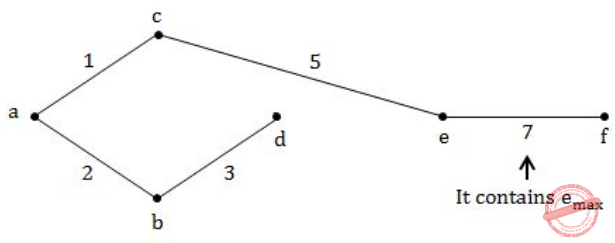Question 127
A list of n strings, each of length n, is sorted into lexicographic order using merge – sort algorithm. The worst case running time of this computation is :
 A O(n log n) B O(n​ 2​ log n) C O(n​ 2​ + log n) D O(n​ 3​ )
Algorithms       Sorting       UGC NET CS 2017 Nov- paper-2
Question 127 Explanation:
1.The comparison is strictly follow the Dictionary based order.
2.The length of the string is n, the time taken is to be O(n) for each comparison.
3. For level -1(bottom-up order): Every element has to be compared with other element the number of comparisons is O(n/2).
4. For level -1(bottom-up order): Total time taken by one level is O(n​ 2​ ).
5. For copying level to level the time taken by O(n​ 2​ ).
So, For level -1= O(n​ 2​ )+ O(n​ 2​ )
6. Second level O(n​ 2​ )+ O(n​ 2​ ).
;
;
;
Final n level (logn)*O(n​ 2​ ) = O(n​ 2​ logn)
 Question 128
Postorder traversal of a given binary search tree T produces following sequence of keys:3, 5, 7, 9, 4, 17, 16, 20, 18, 15, 14 Which one of the following sequences of keys can be the result of an in-order traversal of the tree T?
 A 3, 4, 5, 7, 9, 14, 20, 18, 17, 16, 15 B 20, 18, 17, 16, 15, 14, 3, 4, 5, 7, 9 C 20, 18, 17, 16, 15, 14, 9, 7, 5, 4, 3 D 3, 4, 5, 7, 9, 14, 15, 16, 17, 18, 20
Algorithms       Searching       UGC NET CS 2017 Nov- paper-2
Question 128 Explanation:
We can easily find solutions based on given options because option D given all numbers in ascending order. In Order will print ascending order only.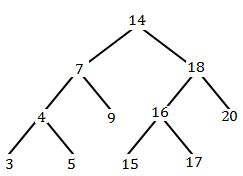Question 129
Let us assume that you construct ordered tree to represent the compound ​ proposition (~ (p ∧ q)) ↔ (~ p ∨ ~ q) Then, the prefix expression and post-fix​ expression determined using this ordered tree are given as ____ and _____ respectively.
 A ↔~∧pq∨ ~ ~ pq, pq∧~p~q~∨↔ B ↔~∧pq∨ ~ p~q, pq∧~p~q~∨↔ C ↔~∧pq∨ ~ ~ pq, pq∧~p~~q∨↔ D ↔~∧pq∨ ~ p~ q, pq∧~p~ ~q∨↔
Algorithms       Trees       UGC NET CS 2016 Aug- paper-2
Question 129 Explanation:
Step-1: Given compound proposition is
(~(p ∧ q))↔(~ p ∨ ~ q)
It is clearly specifying that
↔ is a root
(~(p ∧ q)) is left subtree
(~p ∨ ~q) is right subtree.
Step-2: Finally the tree is looks likeStep-3: Prefix operation traverse through Root,left and Right ↔~∧pq∨ ~ p~q Step-4: Postfix operation traverse through Left,Right and Root.
pq∧~p~q~∨↔
 Question 130
Given i= 0, j = 1, k = –1, x = 0.5, y = 0.0 What is the output of given ‘C’ expression ?
x * 3 && 3 || j | k
 A -1 B 0 C 1 D 2
Algorithms       UGC NET CS 2016 Aug- paper-2
Question 130 Explanation:
Step-1: Evaluate x ​ * 3 because multiplication has more priority than remaining operators x * 3→ 1.5
Step-2: && is logical AND. Both the statements are TRUE, then it returns 1 otherwise 0.
1.5 && 3 is TRUE. So, it returns 1.
Step-3: j | k is bitwise OR operator. It returns -1.
Step-4: ((x * 3) && 3) || (j | k) islogical OR operator. It returns 1
Note: The precedence is ((x * 3) && 3) || (j | k)
 Question 131
The runtime for traversing all the nodes of a binary search tree with n nodes and printing them in an order is:
 A O(lg n) B O(n lg n) C O(n) D O(n​ 2​ )
Algorithms       Searching       UGC NET CS 2016 Aug- paper-2
Question 131 Explanation:
Possibility-1:
→ Traversing all the nodes of a binary search tree with n nodes and printing them in order using inorder traversal.
→ Inorder traversal will print all elements in sorted order. It takes worst case time complexity is O(n).
Possibility-2:
Without sorted elements chance to form either left skewed or right skewed tree. It takes worst case time complexity is O(n).
 Question 132
In general, in a recursive and non-recursive implementation of a problem (program) :
 A Both time and space complexities are better in recursive than in non-recursive program. B Both time and space complexities are better in non-recursive than in recursive program C Time complexity is better in recursive version but space complexity is better in non-recursive version of the program. D Space complexity is better in recursive version but time complexity is better in non-recursive version of the program.
Algorithms       Time-Complexity       UGC NET CS 2015 Dec- paper-2
Question 132 Explanation:
Both time and space complexities are better in non-recursive than in recursive program. We can also call non-recursive programs are iterative programs. Iterative programs time and space complexity is better than recursive program.
→ Recursion uses more memory, but is sometimes clearer and more readable. Using loops increases the performance, but recursion can sometimes be better for the programmer (and his performance). Depends on what we you want to implement, and what's more important for you (readability, performance...)
Example: Factorial of a number.
 Question 133
In the following graph, discovery time stamps and finishing time stamps of Depth First Search (DFS) are shown as x/y, where x is discovery time stamp and y is finishing time stamp.It shows which of the following depth first forest?
 A {a, b, e} {c, d, f, g, h} B {a, b, e} {c, d, h} {f, g} C {a, b, e} {f, g} {c, d} {h} D {a, b, c, d} {e, f, g} {h}
Algorithms       Depth-first-search       UGC NET CS 2015 Dec- paper-2
Question 133 Explanation:
A DFS starting at some vertex ‘v’ explores the graph by building up a tree that contains all vertices that are reachable from ‘v’ and all edges that are used to reach these vertices. We call this tree a DFS tree. A complete DFS exploring the full graph (and not only the part reachable from a given vertex ‘v’) builds up a collection of trees, or forest, called a DFS forest.
Based on definition of DFS forest, option-A is correct.
 Question 134
An ideal sort is an in-place-sort whose additional space requirement is __________.
 A O (log​ 2​ n) B O (n log​ 2​ n) C O (1) D O (n)
Algorithms       Sorting       UGC NET CS 2015 Dec- paper-2
Question 134 Explanation:
The ideal sorting algorithm would have the following properties:
1. Stable: Equal keys aren't reordered.
2. Operates in place, requiring O(1) extra space.
3. Worst case O(nlog(n)) key comparisons.
4. Worst case O(n) swaps.
5. Adaptive: Speeds up to O(n) when data is nearly sorted or when there are few unique keys.
 Question 135A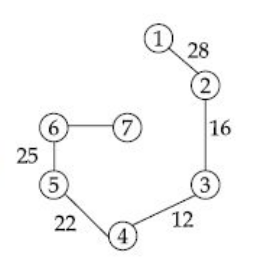BC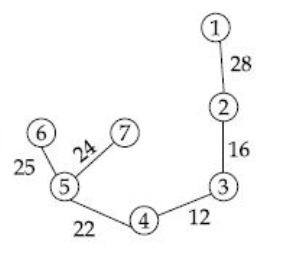D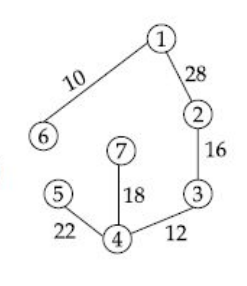Algorithms       Minimum-Spanning-Tree       UGC NET CS 2015 Jun- paper-2
Question 135 Explanation:
To find minimum cost spanning tree, we are using either prim’s algorithm (or) kruskal’s algorithm.
According to kruskal’s algorithm, first we have sort an elements.
Elements are: 10,12,14,16,18,22,24,25,28Question 136
The average case occurs in the linear search algorithm when:
 A The item to be searched is in somewhere middle of the array B The item to be searched is not in the array C The item to be searched is in the last of the array D The item to be searched is either in the last or not in the array
Algorithms       Searching       UGC NET CS 2015 Jun- paper-2
Question 136 Explanation:
→ A linear search or sequential search is a method for finding an element within a list. It sequentially checks each element of the list until a match is found or the whole list has been searched.
→ A linear search runs in at worst linear time and makes at most n comparisons, where ‘n’ is the length of the list. If each element is equally likely to be searched, then linear search has an average case of n/2 comparisons, but the average case can be affected if the search probabilities for each element vary. → Linear search is rarely practical because other search algorithms and schemes, such as the binary search algorithm and hash tables, allow significantly faster searching for all but short lists.
 Question 137
To determine the efficiency of an algorithm the time factor is measured by:
 A Counting microseconds B Counting number of key operations C Counting number of statements D Counting kilobytes of algorithm
Algorithms       Time-Complexity       UGC NET CS 2015 Jun- paper-2
Question 137 Explanation:
To determine the efficiency of an algorithm the time factor is measured by counting number of key operations.
 Question 138
Which of the following algorithms sort n integers, having the range 0 to (n​ 2​ - 1), in ascending order in O(n) time ?
 A Selection sort B Bubble sort C Radix sort D Insertion sort
Algorithms       Sorting       UGC NET CS 2015 Jun- paper-2
Question 138 Explanation:
Consider sorting n integers in the range 0 to n​ 2​ - 1. We do it in two phases.
Phase 1:
1. We use n bins, one for each of the integers 0,1,.., n-1. We place each integer i on the list to be sorted into the bin numbered (i mod n) each bin will then contain a list of integers leaving the same remainder when divided by n.
At the end, we concatenate the bins in order to obtain a list L.
Phase 2:
1. The integers on the list L are redistributed into bins, but using the bin selection
function: ⌊i/n⌋
2. Now append integers to the ends of lists. Finally, concatenate the lists to get the final sorted sequence.
 Question 139
How much extra space is used by heapsort ?
 A O(1) B O(Log n) C O(n) D O(n​ 2​ )
Algorithms       Sorting       UGC NET CS 2004 Dec-Paper-2
Question 139 Explanation:
→ Heap sort uses Max-Heapify function which calls itself but it can be made using a simple while loop and thus making it an iterative function which intern takes no space. So, the space complexity of Heap Sort can be reduced to O(1)
→ The heapify function does not take O(logn) space, it will take O(logn) time in worst case. Here we don't do heapify through any recursion call. We will do through iterative method and we don't require any auxiliary space in this sorting. We use only input array.
So, we do heap sort in O(1) space complexity.
 Question 140
Which algorithm has same average, worst case and best case time ?
 A Binary search B Maximum of n number C Quick sort D Fibonacci search
Algorithms       Time-Complexity       UGC NET CS 2006 Dec-paper-2
Question 140 Explanation: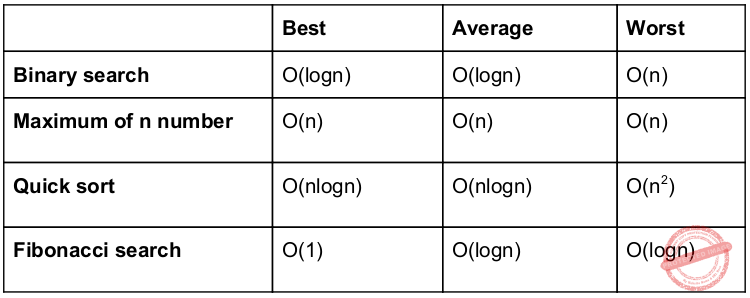Question 141
You have to sort a list L, consisting of a sorted list followed by a few ‘random’ elements. Which of the following sorting method would be most suitable for such a task ?
 A Bubble sort B Selection sort C Quick sort D Insertion sort
Algorithms       Sorting       UGC NET CS 2014 Dec-Paper-2
Question 141 Explanation:
Here, they given sorted list followed by a few ‘random’ elements.
So, we can eliminate option-B and Option-C because it gives worst complexity(O(n​ 2​ )) when array is already sorted.
Bubble sort and insertion sort will give best case complexity(O(n)) but here they given small constraint is “there are few random elements”. So, insertion sort is more appropriate answer.
 Question 142
Big-O estimates for the factorial function and the logarithm of the factorial function i.e. n! and log n! is given by
 A O(n!) and O(n log n) B O(nn) and O(n log n) C O(n!) and O(log n!) D O(nn) and O(log n!)
Algorithms       Time-Complexity       UGC NET CS 2014 June-paper-2
Question 142 Explanation:
→ Given factorial function is n!. we can also write into nn. When we are writing into asymptotic order also the value remains same O(nn).
→ Given logarithm of the factorial function log n!.
= log n! (or)
= log nn (or)
= nlogn
When we are writing into asymptotic order also the value remains same O(nlogn).
 Question 143
The amortized time complexity to perform ______ operation(s) in Splay trees is O(lg n).
 A Search B Search and Insert C Search and Delete D Search, Insert and Delete
Algorithms       Time-Complexity       UGC NET CS 2013 Sep-paper-2
Question 143 Explanation:
→ Amortized analysis is a method for analyzing a given algorithm's complexity, or how much of a resource, especially time or memory, it takes to execute. The motivation for amortized analysis is that looking at the worst-case run time per operation, rather than per algorithm, can be too pessimistic.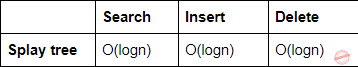Search (or) Find: For the Search (or) Find operation, we perform a normal BST ﬁnd followed by a splay operation on the node found (or the leaf node last encountered, if the key was not found). We can charge the cost of going down the tree to the splay operation. Thus the amortized cost of ﬁnd is O(log n).
Insert/delete: The amortized cost of the splay operation is also O(log n), and thus the amortized cost of insert/delete is O(log n).
 Question 144
Suppose that the splits at every level of Quicksort are in proportion 1-β to β, where 0 < β ≤ 0.5 is a constant. The number of elements in an array is n. The maximum depth is approximately
 A 0.5 β Ig n B 0.5 (1 – β) Ig n C – (Ig n)/(Ig β) D – (Ig n)/Ig (1 – β)
Algorithms       Sorting       UGC NET CS 2013 Sep-paper-2
 Question 145
The solution of the recurrence relation T(m)=T(3m/4)+1 is :
 A θ(lg m) B θ(m) C θ(mlg m) D θ(lglg m)
Algorithms       Asymptotic-Complexity       UGC NET CS 2018 JUNE Paper-2
Question 145 Explanation:
Using Masters Method:
a=1, b=4/3, k=0, p=0
Here, a = b​ k
So, T(m) = n log a/ log b log p+1 n
T(m) = θ(log m)
 Question 146
Which of the following algorithms solves the single-source shortest paths ?
 A Prim’s algorithm B Floyd - Warshall algorithm C Johnson’s algorithm D Dijkstra’s algorithm
Algorithms       Greedy-approach       UGC NET CS 2018 JUNE Paper-2
Question 146 Explanation:
Dijkstra’s algorithm is a Single source shortest path algorithm used to find the shortest path using Greedy approach.
 Question 147
A text is made up of the characters A, B, C, D, E each occurring with the probability 0.08, 0.40, 0.25, 0.15 and 0.12 respectively. The optimal coding technique will have the average length of :
 A 2.4 B 1.87 C 3 D 2.15
Algorithms       Greedy-approach       UGC NET CS 2018 JUNE Paper-2
Question 147 Explanation:Step 1: Select two characters with smallest probabilities and merge them.Step 2: Select two characters from Step 1 with smallest probabilities and merge them.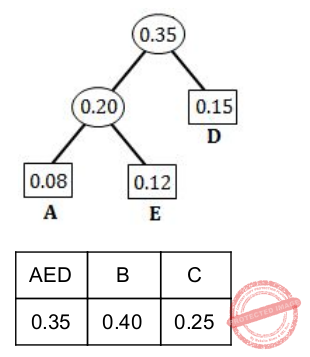Step 3: Select two characters (from Step 2) with smallest probabilities and merge them.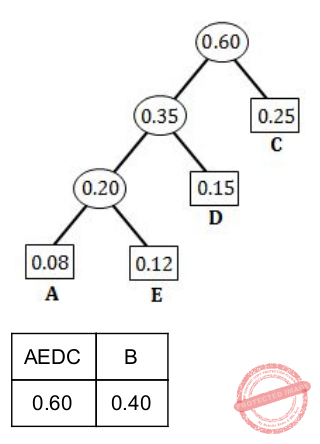Step 4: Merge the remaining two probabilities.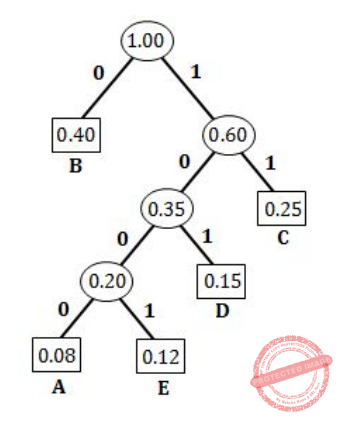A = 1000 (4-bits)
E = 1001 (4-bits)
D = 101 (3-bits)
C = 11 (2-bits)
B = 0 (1-bit)
Average length = ((0.08×4)+(0.12×4)+(0.15×3)+(0.25×2)+(0.40×1)) / (0.08+0.12+0.15+0.25+0.40)
=2.15/1.0
= 2 .15
 Question 148
Match the following with respect to algorithm paradigms :A (a)-(iv),(b)-(i), (c)-(iii), (d)-(ii) B (a)-(iv),(b)-(iii), (c)-(i), (d)-(ii) C (a)-(iii),(b)-(iv), (c)-(ii), (d)-(i) D (a)-(iv),(b)-(iii), (c)-(ii), (d)-(i)
Algorithms       Dynamic-Programming-and-Greedy-approach       UGC NET CS 2018 JUNE Paper-2
Question 148 Explanation:
8-Queens problem use Backtracking
Single-Source shortest paths use Greedy approach
STRASSEN’s Matrix multiplication use Divide and conquer technique
Optimal binary search trees use Dynamic programming
 Question 149
The maximum number of comparisons needed to sort 9 items using radix sort is (assume each item is 5 digit octal number) :
 A 45 B 72 C 360 D 450
Algorithms       Sorting       UGC NET CS 2018 JUNE Paper-2
Question 149 Explanation:
Total sort items=9
Octal number having→ 5 digits
The octal number system base value= 8
The maximum number of comparison=(number of items)*(radix)*(number of digits)
= 9*5*8
= 360
 Question 150
Big – O estimate for f(x) = (x+1) log(x2+1)+3x2 is given as
 A O(x log x) B O(x2) C O(x3) D O(x2 log x)
Algorithms       Asymptotic-Complexity       UGC NET CS 2013 Dec-paper-2
Question 150 Explanation:
f(x) = (x+1) log(x2+1)+3x2 in this function, 3x2 is leading term. So, we can call asymptotically O(n2).
 Question 151
The total number of comparisons in a bubble sort is
 A 0(log n) B 0(n log n) C 0(n) D None of the above
Algorithms       Sorting       UGC NET CS 2011 Dec-Paper-2
Question 151 Explanation:
The total number of comparisons in a bubble sort is O(n2)
 Question 152
Which of the following is not primitive recursive but computable ?
 A Carnot function B Riemann function C Bounded function D Ackermann function
Algorithms       Ackermann-function       NIELIT Technical Assistant_2016_march
 Question 153
Give as good a big–O estimate as possible for the following functions : (nlogn+n2)(n3+2) and (n!+2n)(n3+log(n2+1))
 A O(n5+2n2) & O(n3*n!) B O(n5) & O(n3*2n) C O(n5) & O(n3* n!) D O(n5+2n2) & O(n3*2n)
Algorithms       Asymptotic-Complexity       UGC NET CS 2013 June-paper-2
Question 153 Explanation:
Step-1: Consider first function (nlogn+n2)(n3+2).
Here, It is performing multiplication of 2 polynomials.
(nlogn+n2) → Raising value is n2. We can write asymptotically O(n2)
(n3+2) → Raising value is n3. We can write asymptotically O(n3)
= O(n2)*O(n3)
= O(n5)
Step-2: Second function (n!+2n)(n3+log(n2+1))
Here, It is performing multiplication of 2 polynomials.
(n!+2n) → Raising value is n!. We can write asymptotically O(n!)
(n3+log(n2+1)) → Raising value is n3. We can write asymptotically O(n3)
= O(n!)*O(n3)
= O(n3* n!)
 Question 154
Which of the following connected simple graph has exactly one spanning tree ?
 A Complete graph B Hamiltonian graph C Euler graph D None of the above
Algorithms       Minimum-Spanning-Tree       UGC NET CS 2013 June-paper-2
Question 154 Explanation:
→ Complete graph have nn-2 spanning trees.
→ Hamiltonian graph will get more than one spanning tree.
→ Euler graph will get more than one spanning tree.
 Question 155
The total number of spanning trees that can be drawn using five labelled vertices is :
 A 125 B 64 C 36 D 16
Algorithms       Minimum-Spanning-Tree       UGC NET CS 2008 Dec-Paper-2
Question 155 Explanation:
To find total number of spanning trees we are using a standard formula is nn-2.
n=5
=55-2
=53
=125
 Question 156
The complexity of Kruskal’s minimum spanning tree algorithm on a graph with ‘n’ nodes and ‘e ’ edges is :
 A O (n) B O (n log n) C O (e log n) D O (e)
Algorithms       Minimum-Spanning-Tree       UGC NET CS 2008-june-Paper-2
Question 156 Explanation:
MST-KRUSKAL(G,w)
1. A=∅
2. for each vertex v∈ G.V
3. MAKE-SET(v)
4. sort the edges of G.E into nondecreasing order by weight w
5. for each edge (u,v)∈G.E, taken in nondecreasing order by weight
6. if FIND-SET(u)≠FIND-SET(v)
7. A=A∪{(u,v)}
8. UNION(u,v)
9. return A
Analysis:
The running time of Kruskal’s algorithm for a graph G=(V,E) depends on how we implement the disjoint-set data structure.
→ Initializing the set A in line 1 takes O(1) time, and the time to sort the edges in line 4 is O(ElgE). (We will account for the cost of the |V| MAKE-SET operations in the for loop of lines 2–3 in a moment.)
→ The for loop of lines 5–8 performs O(E) FIND-SET and UNION operations on the disjoint-set forest.
→ Along with the |V| MAKE-SET operations, these take a total of O((V+E)α(V)) time, where α is the very slowly growing function.
→ Because we assume that G is connected, we have |E|>=|V|-1, and so the disjoint-set operations take O(Eα(V)) time.
→ Moreover, since α |V|=O(lg V)=O(lg E), the total running time of Kruskal’s algorithm is O(ElgE)
→ Observing that |E|<|V|2 , we have lg |E|=O(lg V), and so we can restate the running time of Kruskal’s algorithm as O(E lg V)
 Question 157
Which of the following is true for a sorted list with ‘n’ elements ?
 A Insertion in a sorted array takes constant time. B Insertion in a sorted linear linked list takes constant time. C Searching for a key in a sorted array can be done in O(log n) time. D Searching for a key in a sorted linear linked list can be done in O(log n) time.
Algorithms       Sorting       UGC NET CS 2008-june-Paper-2
Question 157 Explanation:
TRUE: Searching for a key in a sorted array can be done in O(log n) time.
Because elements are in sorted order, we are using binary search tree. it will take worst case time complexity O(logn) only.
 Question 158
The time required to find shortest path in a graph with n vertices and e edges is :
 A O(e) B O(n) C O(e2) D O(n2)
Algorithms       Greedy-approach       UGC NET CS 2007 June-Paper-2
Question 158 Explanation:
→ We can use dijkstra’s algorithm to find shortest path in a graph with n vertices and e edges is O(ElogV).
→ We know that E ≤ V2 if graph is complete and time complexity is O(n2)
 Question 159
Which algorithm has some average, worst case and best case time :
 A Binary search B Maximum of n numbers C Quick sort D Fibonacci search
Algorithms       Time-Complexity       UGC NET CS 2007 June-Paper-2
Question 159 Explanation: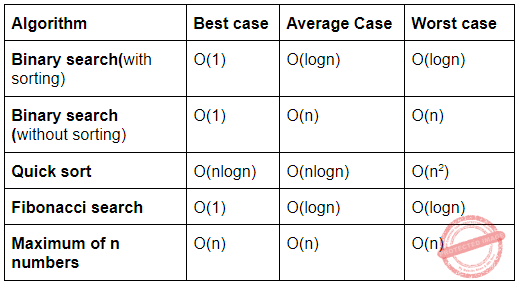Question 160
You are given a sequence of n elements to sort. The input sequence consists of n/k subsequences,each containing k elements. The elements in a given subsequence are all smaller than the elements in the succeeding subsequence and larger than the elements in the preceding subsequence. Thus, all that is needed to sort the whole sequence of length n is to sort the k elements in each of the n/k subsequences. The lower bound on the number of comparisons needed to solve this variant of the sorting problem is
 A Ω(n) B Ω(n/k) C Ω(nlogk ) D Ω(n/klogn/k)
Algorithms       Sorting       UGC NET CS 2017 Nov- paper-3
Question 160 Explanation:
There are n/k subsequences and each can be ordered in k! ways. This makes a (k!)n/k outputs. We can use the same reasoning:
(k!)n/k ≤ 2h
Taking the logarithm of both sides, we get:
h ≥ lg(k!)n/k
= (n/k)lg(k!)
≥ (n/k)(k/2)lg(k/2)
= (1/2)*(nlogk)-(1/2)*n
= Ω(nlogk)
 Question 161
T(n)= 8T (n/2)+Cn, if n>1 = b, if n=1 Consider the recurrence relation:   Where b and c are constants. The order of the algorithm corresponding to above recurrence relation is:
 A n B n2 C n log n D n3
Algorithms       Time-Complexity       UGC NET CS 2017 Nov- paper-3
Question 161 Explanation:
The above recurrence is in the form of masters theorem.
a=8,b=2,k=0 and p=0
Case-1: a>bk = 8>20
T(n)=O(nlogb^a)
= O(n3)
 Question 162
Consider the following two sequences :
X = < B, C, D, C, A, B, C > and
Y = < C, A, D, B, C, B >
The length of longest common subsequence of X and Y is :
 A 5 B 3 C 4 D 2
Algorithms       Dynamic-Programming       UGC NET CS 2017 Nov- paper-3
Question 162 Explanation: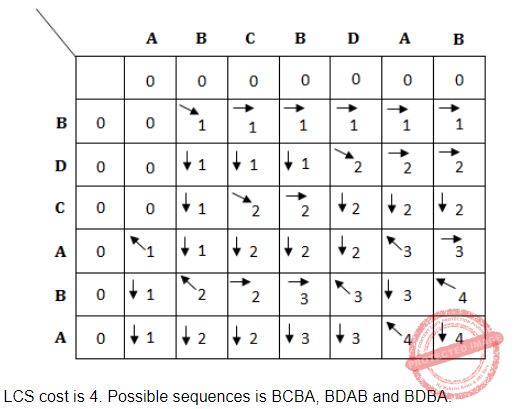Question 163
A text is made up of the characters a, b, c, d, e each occurring with the probability 0.11, 0.40, 0.16, 0.09 and 0.24 respectively. The optimal Huffman coding technique will have the average length of:
 A 2.4 B 2.16 C 2.26 D 2.15
Algorithms       Greedy-approach       UGC NET CS 2017 Nov- paper-3
Question 163 Explanation: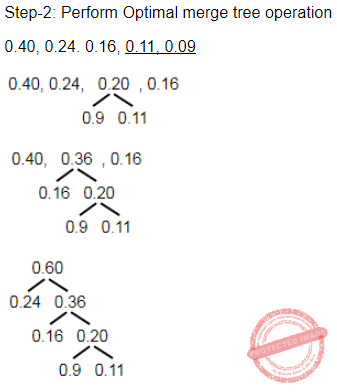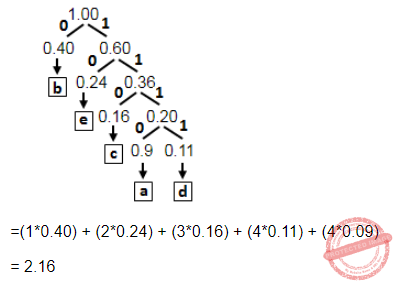Question 164
An undirected graph G (V, E) contains n (n > 2) nodes named v1 , v2 ,...,vn. Two nodes vi and vj are connected if and only if 0 <│i−j│≤2. Each edge (vi , vj ) is assigned a weight i+j. The cost of the minimum spanning tree of such a graph with 10 nodes is:
 A 88 B 91 C 49 D 21
Algorithms       Minimum-Spanning-Tree       UGC NET CS 2017 Nov- paper-3
Question 164 Explanation:
Method-1: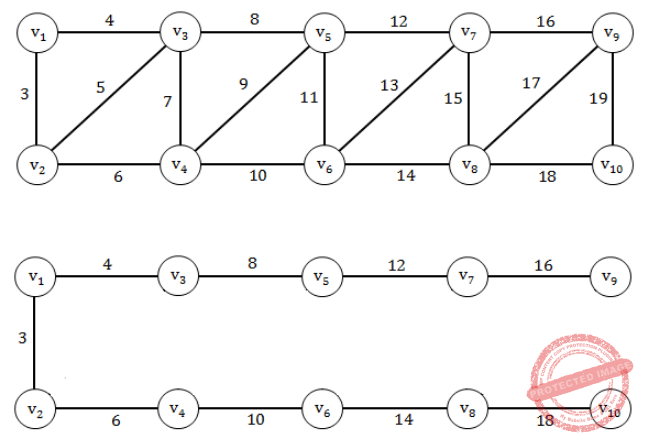Question 165
If b is the branching factor and m is the maximum depth of the search tree, what is the space complexity of greedy search?
 A O(b+m) B O(bm) C O(bm) D O(mm)
Algorithms       Greedy-approach       UGC NET CS 2017 Nov- paper-3
Question 165 Explanation:Question 166
Any decision tree that sorts n elements has height ________.
 A Ω(lg n) B Ω(n) C Ω(n lg n) D Ω(n2)
Algorithms       Sorting       UGC NET CS 2017 Jan- paper-3
Question 166 Explanation:
Theorem: Any decision tree that sorts n elements has height Ω(n lg n).
Proof:
→ The number of leaves l ≥ n!
→ Any binary tree of height h has ≤ 2h leaves.
→ Hence, n! ≤ l ≤ 2h
→ Take logs: h ≥ lg(n!)
→ Use Stirling’s approximation: n! > (n/e)n
→ We get:
h ≥ log(n/e)n
= nlg(n/e)
= nlg n - n lg e
= Ω(n lg n)
 Question 167
Red-black trees are one of many search tree schemes that are “balanced” in order to guarantee that basic dynamic-set operations take ________ time in the worst case.
 A O(1) B O(lg n) C O(n) D O(n lg n)
Algorithms       Algorithms Time-Complexity       UGC NET CS 2017 Jan- paper-3
 Question 168
The minimum number of scalar multiplication required, for parenthesization of a matrix-chain product whose sequence of dimensions for four matrices is <5, 10, 3, 12, 5> is
 A 630 B 580 C 480 D 405
Algorithms       Dynamic-Programming       UGC NET CS 2017 Jan- paper-3
Question 168 Explanation:Question 169
Dijkstra’s algorithm is based on
 A Divide and conquer paradigm B Dynamic programming C Greedy Approach D Backtracking paradigm
Algorithms       Greedy-approach       UGC NET CS 2017 Jan- paper-3
Question 169 Explanation:
Dijkstra’s algorithm is used on greedy approach for single source shortest path problem.
 Question 170
Match the following with respect to algorithm paradigms : 36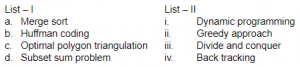A a-iii, b-i, c-ii, d-iv B a-ii, b-i, c-iv, d-iii C a-ii, b-i, c-iii, d-iv D b-iii, b-ii, c-i, d-iv
Algorithms       Sorting       UGC NET CS 2017 Jan- paper-3
Question 170 Explanation:
Merge sort→ Divide and conquer
Huffman coding→ Greedy approach
Optimal polygon triangulation→ Dynamic programming
Subset sum problem → Backtracking
Note: We can also use subset sum problem as well backtracking.
 Question 171
Consider the problem of a chain < A1, A2, A3, A4 > of four matrices. Suppose that the dimensions of the matrices A1, A2, A3 and A4 are 30 × 35, 35 × 15, 15 × 5 and 5 × 10 respectively. The minimum number of scalar multiplications needed to compute the product A1A2A3A4 is ____.
 A 14875 B 21000 C 9375 D 11875
Algorithms       Dynamic-Programming       UGC NET CS 2016 Aug- paper-3
Question 171 Explanation:Question 172
Consider a weighted complete graph G on the vertex set {ν1, ν2, .... νn} such that the weight of the edge (νi, νj) is 4 | i – j|. The weight of minimum cost spanning tree of G is :
 A 4n2 B n C 4n-4 D 2n-2
Algorithms       Minimum-Spanning-Tree       UGC NET CS 2016 Aug- paper-3
Question 172 Explanation:
1) Including the initial call which means write the length of the spanning tree, a simple one it is. (Based on a particular graph)
2)Weight of the minimum spanning tree = 4|2 - 1| + 4|3 - 2| + 4|4 - 3| + 4|5 - 4| .... + 4| n - (n-1) | = 4n - 2
 Question 173
If there are n integers to sort, each integer has d digits, and each digit is in the set {1, 2, ...,k}, radix sort can sort the numbers in:
 A O (k (n + d)) B O(d (n + k)) C O((n + k) lg d) D O((n + d) lg k)
Algorithms       Sorting       UGC NET CS 2016 Aug- paper-3
Question 173 Explanation: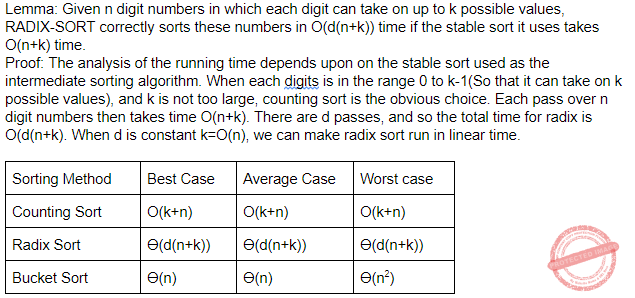Question 174
Match the following:A a-i, b-iii, c-iv, d-ii B a-i, b-iii, c-ii, d-iv C a-iii, b-i, c-iv, d-ii D a-iii, b-i, c-ii, d-iv
Algorithms       Greedy-approach       UGC NET CS 2016 Aug- paper-3
Question 174 Explanation:
Prim’s algorithm→ O(ElogV)
Bellman-Ford algorithm→ O(V2E)
Floyd-Warshall algorithm→ O(V3)
Johnson’s algorithm→ O(VE lg V)
 Question 175
Given two sequences X and Y :

X = < a, b, c, b, d, a, b >

Y = < b, d, c, a, b, a >

The longest common subsequence of X and Y is :

 A < b, c, a > B < c, a, b > C < b, c, a, a > D < b, c, b, a >
Algorithms       Dynamic-Programming       UGC NET CS 2015 Dec - paper-3
Question 175 Explanation:Question 176
If there are n integers to sort, each integer has d digits and each digit is in the set {1, 2, ..., k}, radix sort can sort the numbers in :
 A O(d n k) B O(d nk) C O((d +n) k) D O(d (n + k))
Algorithms       Sorting       UGC NET CS 2015 Dec - paper-3
Question 176 Explanation:
The radix sort running time depends on the stable sort used as the intermediate sorting algorithm. When each digit is in the range 0 to k-1(It takes k possible values), and ‘k’ is not too large, counting sort is the obvious choice. Each pass over n d-digits numbers then takes time O(n+k). There are ‘d’ passes, and so total time for radix sort is O(d (n + k))
 Question 177
The solution of the recurrence relationA O(lg n) B O(n) C O(n lg n) D None of the above
Algorithms       Asymptotic-Complexity       UGC NET CS 2015 Dec - paper-3
Question 177 Explanation:
Assume T(n) = O(1) for small n≤80.
T(n) ≤ T(n/5)+T((7n/10)+6)+O(n)
Inductively verify that T(n)≤cn for some constant c.
T(n) ≤ c(n/5)+c(7n/10+6)+O(n)
≤ 9cn/10+6c+O(n)
≤ cn
In above, choose c so that c((n/10)−6) beats the function O(n) for all n.
Note: They given ‘s’ instead 5.
 Question 178
Floyd-Warshall algorithm utilizes __________ to solve the all-pairs shortest paths problem on a directed graph in __________ time.
 A Greedy algorithm, θ (V3) B Greedy algorithm, θ (V2 lgn) C Dynamic programming, θ (V3) D Dynamic programming, θ (V2 lgn)
Algorithms       Sorting       UGC NET CS 2015 Dec - paper-3
Question 178 Explanation:
Floyd-Warshall algorithm utilizes Dynamic Programming to solve the all-pairs shortest paths problem on a directed graph in θ(V3) time, where “V” is the number of vertices.
There are 178 questions to complete.